SLVSB35C October   2012  – July 2015

PRODUCTION DATA.

1. Features
2. Applications
3. Description
4. Revision History
5. Device Comparison Table
6. Pin Configuration and Functions
7. Specifications
8. Detailed Description
1. 8.1 Overview
2. 8.2 Functional Block Diagrams
3. 8.3 Feature Description
4. 8.4 Device Functional Modes
9. Application and Implementation
1. 9.1 Application Information
2. 9.2 Typical Application
1. 9.2.1 Design Requirements
2. 9.2.2 Detailed Design Procedures
3. 9.2.3 Application Curves
3. 9.3 System Examples
10. 10Power Supply Recommendations
11. 11Layout
12. 12Device and Documentation Support
13. 13Mechanical, Packaging, and Orderable Information

#### Package Options

Refer to the PDF data sheet for device specific package drawings

• DQC|10

## 9 Application and Implementation

NOTE

Information in the following applications sections is not part of the TI component specification, and TI does not warrant its accuracy or completeness. TI’s customers are responsible for determining suitability of components for their purposes. Customers should validate and test their design implementation to confirm system functionality.

### 9.1 Application Information

The TPS6217x is a high-efficiency synchronous step-down DC-DC converter, based on the DCS-Control topology.

With a wide operating input voltage range of 4.75 V to 28 V, the device is ideally suited for systems powered from multi cell Li-Ion as well as 12 V and even higher intermediate supply rails, providing up to 500-mA output current.

### 9.2 Typical Application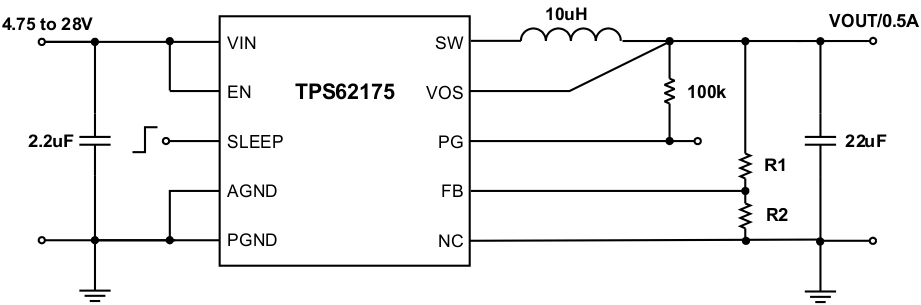Figure 8. Adjustable 0.5-A Power Supply

#### 9.2.1 Design Requirements

The device operates for an input voltage range of 4.75 V to 28 V. The output voltage is adjustable, using an external resistive divider, or internally fixed.

The graphs were generated using the setup according to Figure 8. Table 1 shows the list of components used for the setup.

### Table 1. List of Components

REFERENCE DESCRIPTION MANUFACTURER
IC 28 V, 0.5-A Step-Down Converter, WSON TPS62175DQC, Texas Instruments
L1 10 uH, (4 × 4 × 1.2) mm LPS4012, Coilcraft
Cin 2.2 µF, 50 V, Ceramic, 0805, X5R Standard
Cout 22 µF, 6.3 V, Ceramic, 0805, X5R Standard
R1 depending on VOUT
R2 depending on VOUT
R3 100 kΩ, Chip, 0603, 1/16 W, 1% Standard

#### 9.2.2.1 Programming the Output Voltage

While the output voltage of the TPS62175 is adjustable, the TPS62177 is programmed to a fixed output voltage of 3.3 V. For the fixed output voltage version, the FB pin is pulled low internally by a 400-kΩ resistor. TI recommends connecting the FB pin to AGND to improve thermal resistance. The adjustable version can be programmed for output voltages from 1 V to 6 V by using a resistive divider. The voltage at the FB pin is regulated to 800 mV. The value of the output voltage is set by the selection of the resistive divider from Equation 5. TI recommends choosing resistor values that allow a current of at least 5 uA. Lower resistor values are recommended to increase noise immunity. For applications requiring lowest current consumption, the use of the fixed-output voltage version is recommended.

Equation 5.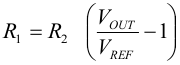As a safety feature, the device clamps the output voltage at the VOS pin to typically 7.4 V, if the FB pin gets opened.

#### 9.2.2.2 External Component Selection

The external components must fulfill the needs of the application, but also the stability criteria of the device's control loop. The TPS6217x is optimized to work within a wide range of external components. The LC output filter's inductance and capacitance must be considered together, creating a double pole that is responsible for the corner frequency of the converter. Table 2 shows the recommended output filter components.

### Table 2. Recommended LC Output Filter Combinations(1)

10 µF 22 µF 47 µF 100 µF 200 µF 400 µF
6.8 µH
10 µH (2)
22 µH
33 µH
(1) The values in the table are nominal values. Variations of typically ±20% due to tolerance, saturation and DC bias are assumed.
(2) This LC combination is the standard value and recommended for most applications. For output voltages of ≤2 V, TI recommends an output capacitance of at least 2 × 22 uF.

#### 9.2.2.2.1 Output Filter and Loop Stability

The TPS6217x devices are internally compensated and are stable with LC output filter combinations recommended in Table 2. Further information on other values and loop stability can be found in Optimizing the TPS62175 Output Filter (SLVA543).

#### 9.2.2.2.2 Inductor Selection

The inductor selection is determined by several effects like inductor ripple current, output ripple voltage, PWM-to-Power Save Mode transition point and efficiency. In addition, the inductor selected must be rated for appropriate saturation current and DC resistance (DCR). Equation 6 and Equation 7 calculate the maximum inductor current under static load conditions.

Equation 6.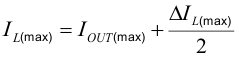spacing

Equation 7.where

• ΔIL is the peak to peak inductor ripple current
• η is the converter efficiency (see efficiency figures)
• L(min) is the minimum inductor value
• fSW is the actual PWM switching frequency

Calculating the maximum inductor current using the actual operating conditions gives the minimum saturation current of the inductor needed. TI recommends a margin of about 20% to cover possible load transient overshoot. A larger inductor value is also useful to get lower ripple current, but increases the transient response time and solution size as well. The inductors listed in Table 3 have been tested with the TPS6217x.

### Table 3. List of Inductors

TYPE INDUCTANCE (µH]) CURRENT (A)(1) DCR (mΩ) DIMENSIONS (LENGTH x WIDTH x HEIGHT) mm MANUFACTURER
LPS4012-103MLC 10 µH, ±20% 1.1 350 (maximum) 4 x 4 × 1.2 Coilcraft
LPS4018-103MLC 10 µH, ±20% 1.3 200 (maximum) 4 x 4 × 1.8 Coilcraft
VLS4012ET-100M 10 µH, ±20% 0.99 190 (typical) 4 x 4 × 1.2 TDK
VLCF4020T-100MR85 10 µH, ±20% 0.85 168 (typical) 4 × 4 × 2 TDK
74437324100 10 µH, ±20% 1.5 215 (typical) 4.5 × 4.1 × 1.8 Wuerth
744025100 10 µH, ±20% 1 190 (maximum) 2.8 × 2.8 × 2.8 Wuerth
IFSC-1515AH-01 10 µH, ±20% 1.3 135 (typical) 3.8 × 3.8 × 1.8 Vishay
ELL-4LG100MA 10 µH, ±20% 0.8 200 (typical) 3.8 × 3.8 × 1.8 Panasonic
(1) IRMS at 40°C rise or ISAT at 30% drop.

#### 9.2.2.2.3 Output Capacitor Selection

The recommended value for the output capacitor is 22 uF. To maintain low output voltage ripple during large load transients, for output voltages less than 2 V, TI recommends 2 × 22 µF output capacitors. The architecture of the TPS6217x allows the use of ceramic output capacitors with low equivalent series resistance (ESR). These capacitors provide low output voltage ripple and are recommended with an X7R or X5R dielectric. Larger capacitance values have the advantage of smaller output voltage ripple and a tighter DC output accuracy in power save mode.

NOTE

In power save mode, the output voltage ripple and accuracy depends on the output capacitance and the inductor value. The larger the capacitance the lower the output voltage ripple and the better the output voltage accuracy. The same relation applies to the inductor value.

#### 9.2.2.2.4 Input Capacitor Selection

Typically, 2.2 µF is sufficient and is recommended, though a larger value reduces input current ripple further. The input capacitor buffers the input voltage during transient events and also decouples the converter from the supply. TI recommends a low ESR, multilayer, X5R or X7R dielectric, ceramic capacitor for best filtering, which should be placed between VIN and PGND as close as possible to those pins.

spacing

spacing

spacing

NOTE

DC Bias effect: High capacitance ceramic capacitors have a DC Bias effect, which has a strong influence on the final effective capacitance. Therefore, the right capacitor value must be chosen carefully. Package size and voltage rating in combination with dielectric material are responsible for differences between the rated capacitor value and the effective capacitance.

#### 9.2.3 Application Curves

VIN=12 V, VOUT = 3.3 V, TJ=25°C, unless otherwise noted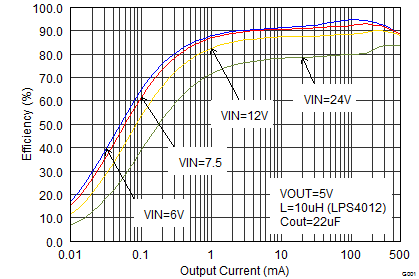Figure 9. Efficiency vs Load Current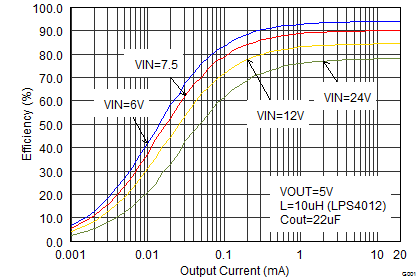Figure 11. Efficiency vs Load Current (Sleep Mode)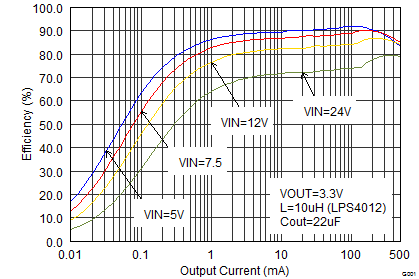Figure 13. Efficiency vs Load Current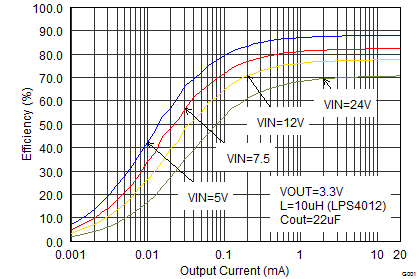Figure 15. Efficiency vs Load Current (Sleep Mode)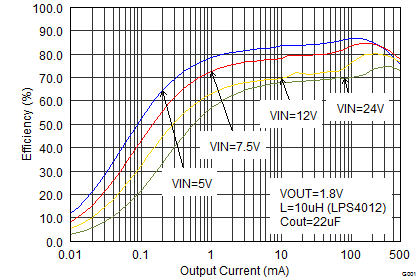Figure 17. Efficiency vs Load Current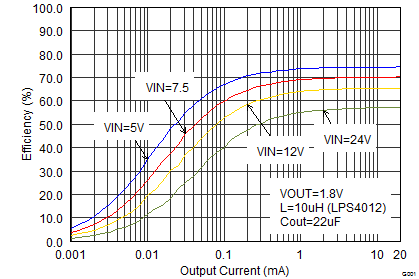Figure 19. Efficiency vs Load Current (Sleep Mode)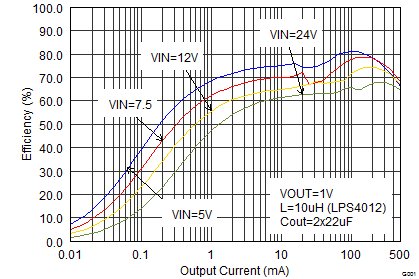Figure 21. Efficiency vs Load Current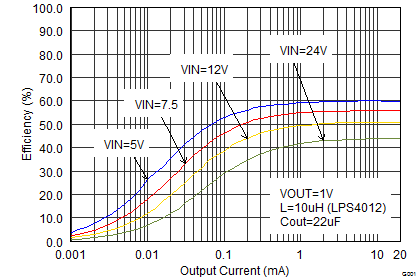Figure 23. Efficiency vs Load Current (Sleep Mode)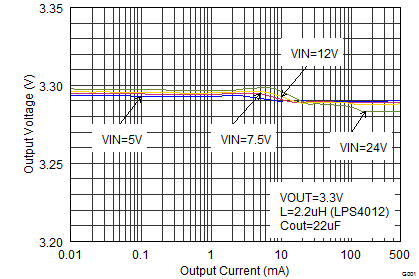Figure 25. Output Voltage Accuracy (Load Regulation)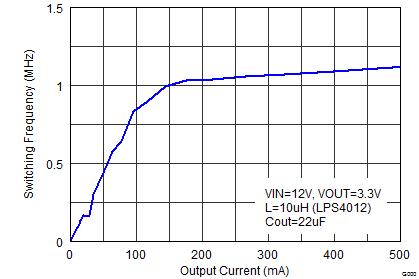Figure 27. Switching Frequency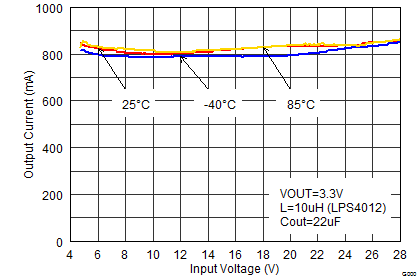Figure 29. Maximum Output Current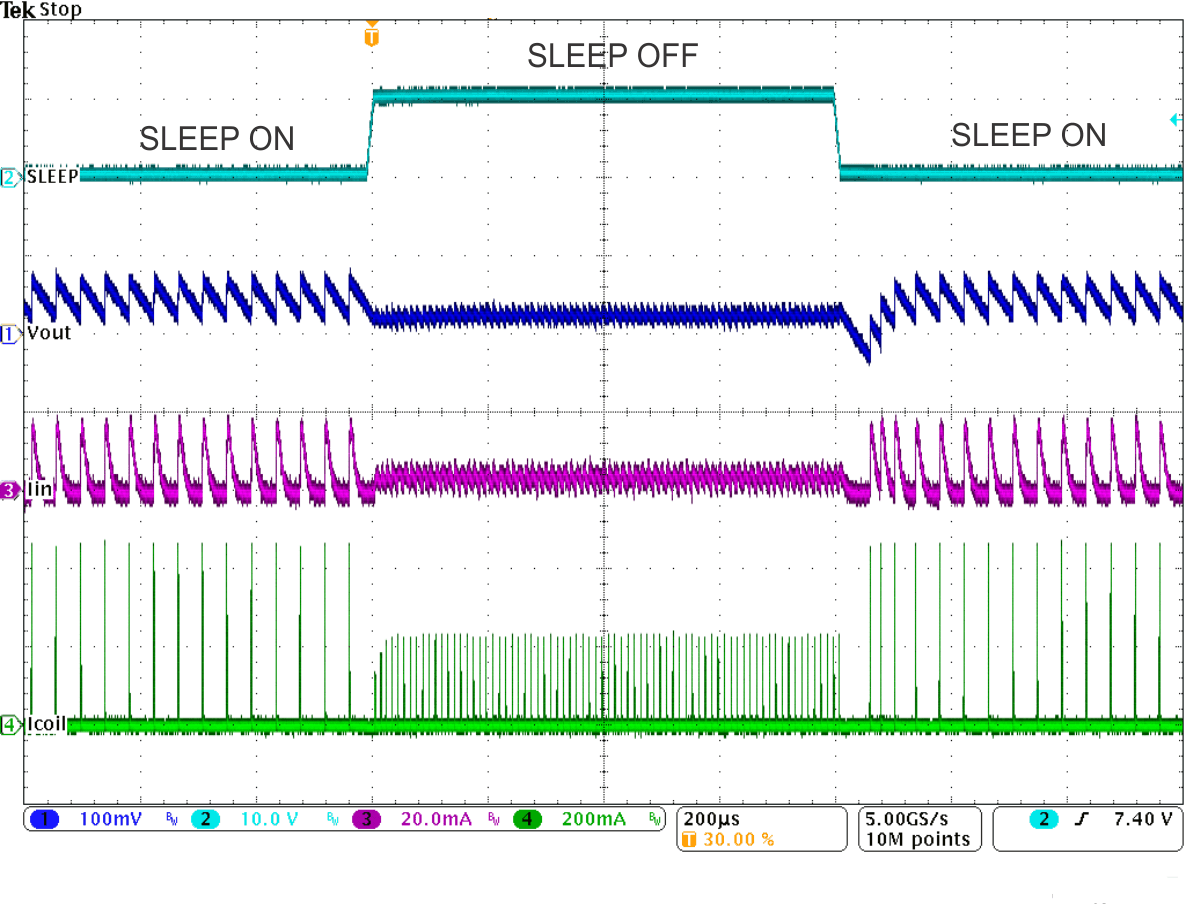Figure 31. Sleep Mode Entry/Exit, IOUT = 10 mA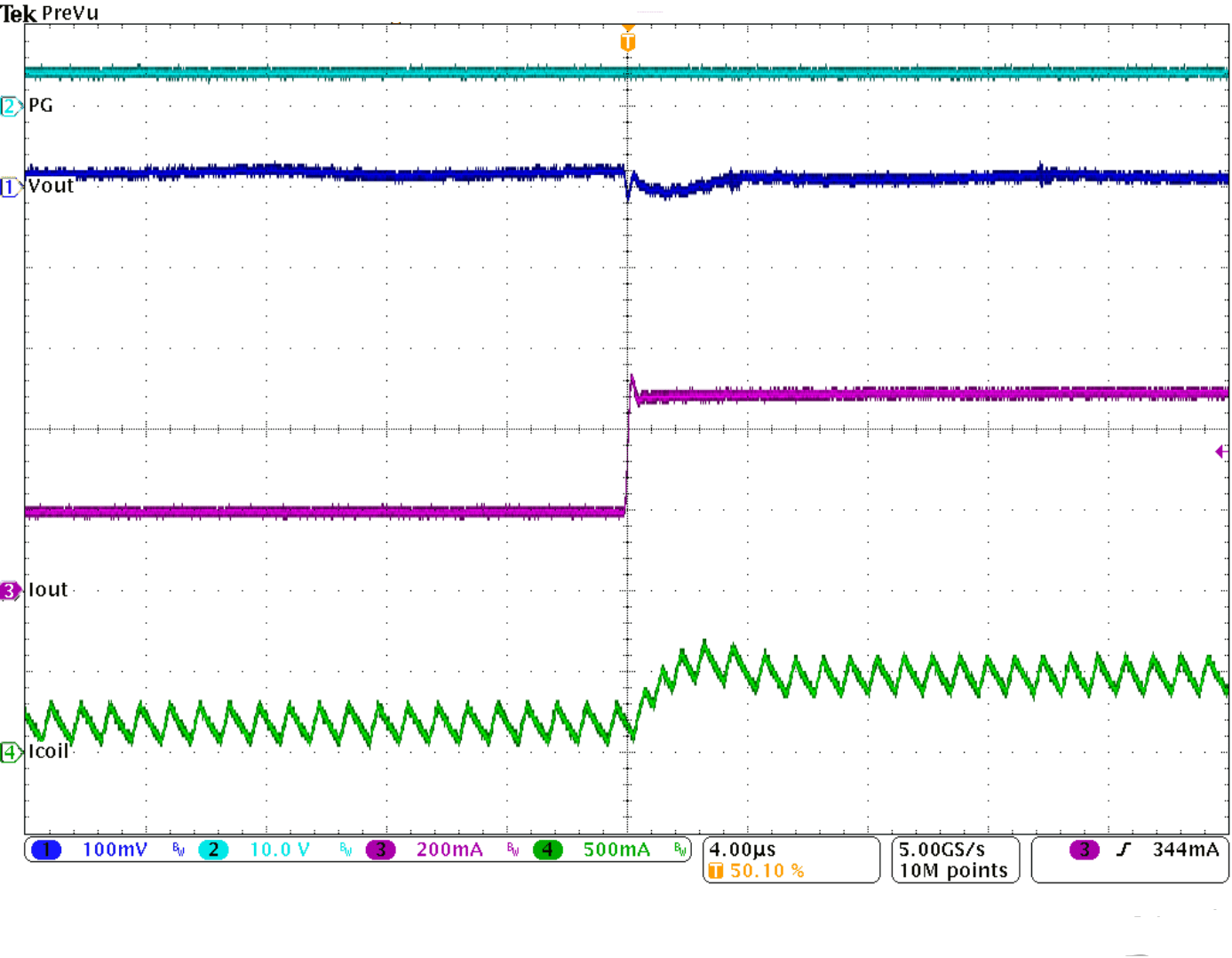Figure 33. Load Transient Response, PWM Mode, IOUT (200 mA to 500 mA), Rising Edge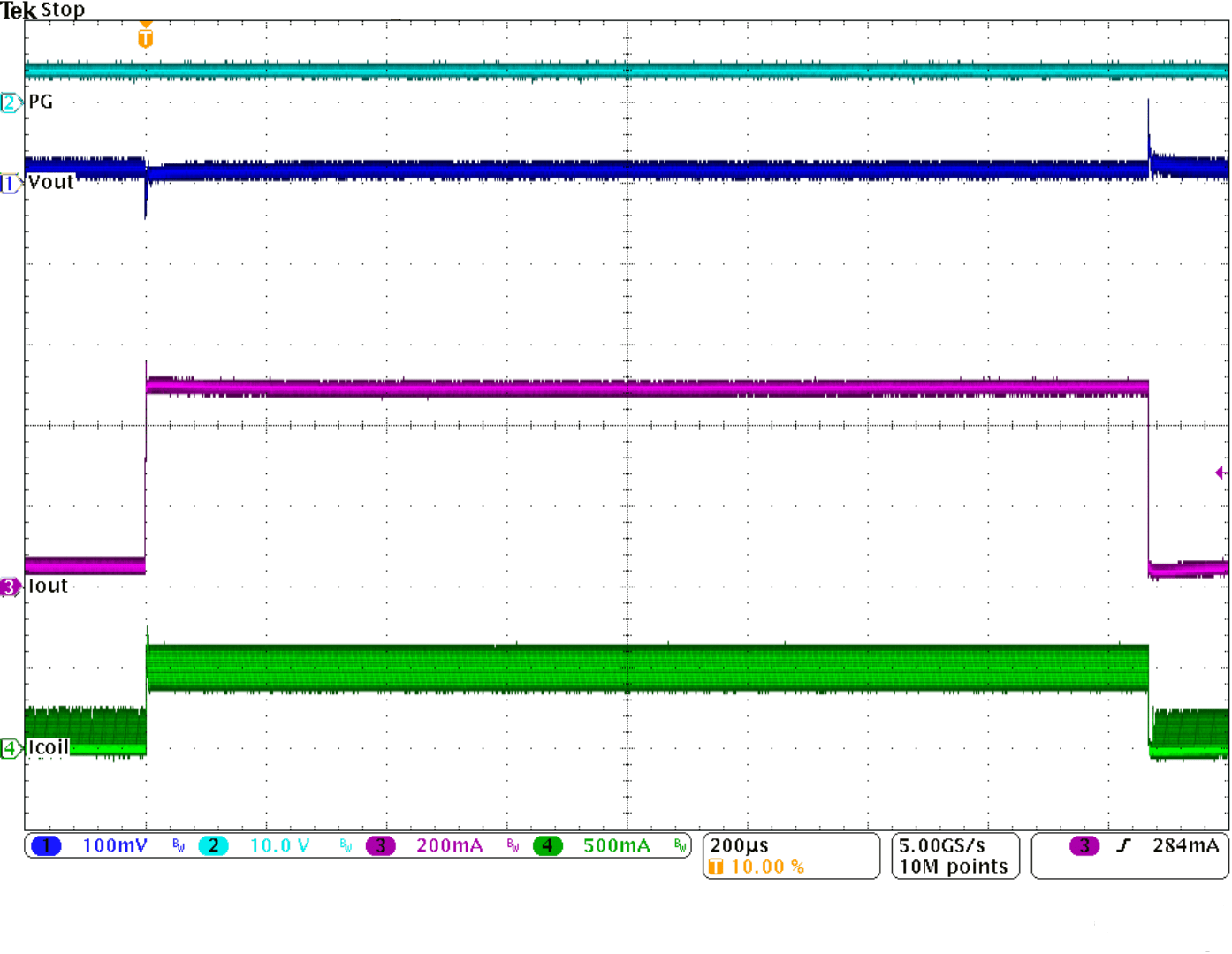Figure 35. Load Transient Response, Power Save Mode, IOUT (50 mA to 500 mA)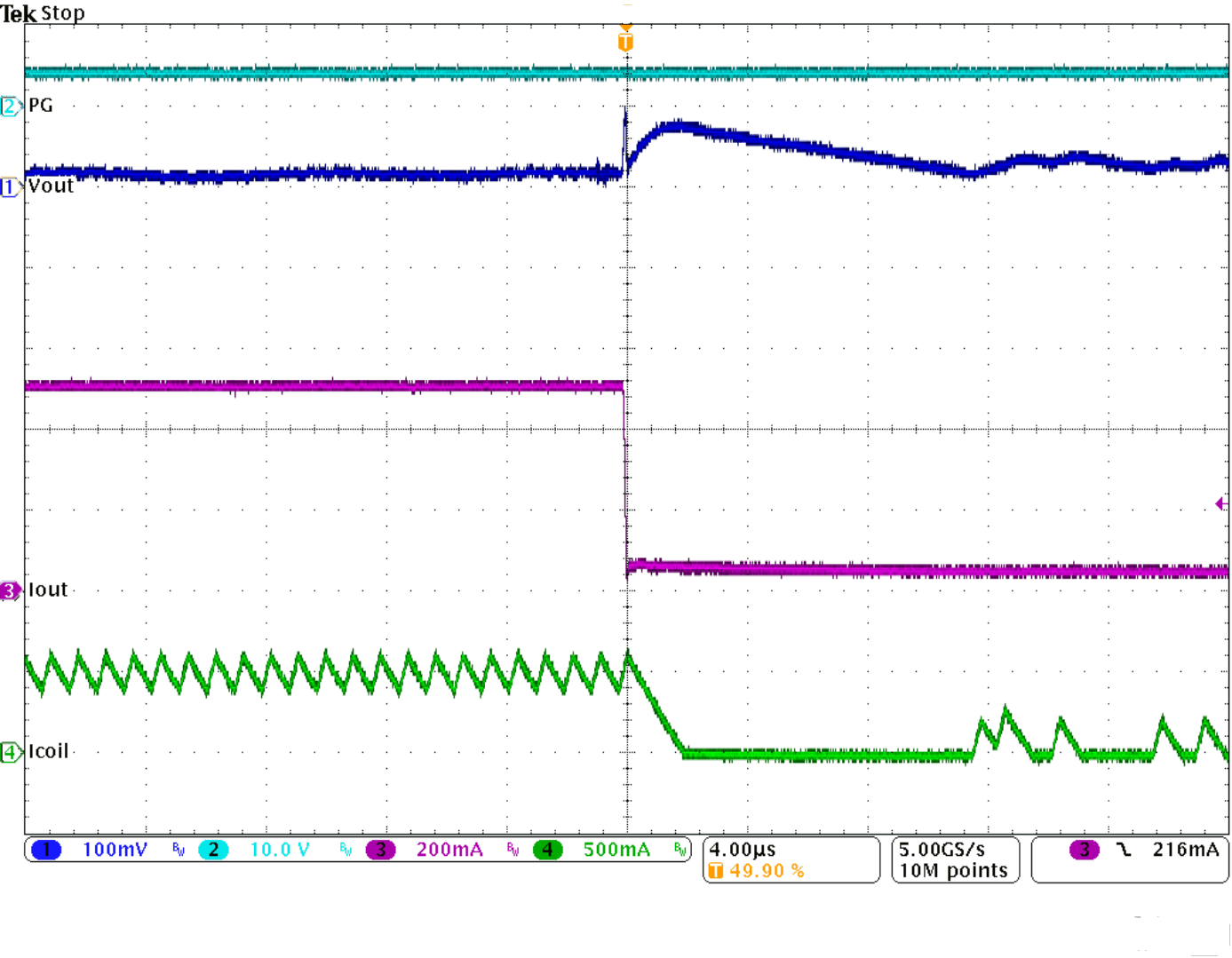Figure 37. Load Transient Response, Power Save Mode, IOUT (50 mA to 500 mA), Falling Edge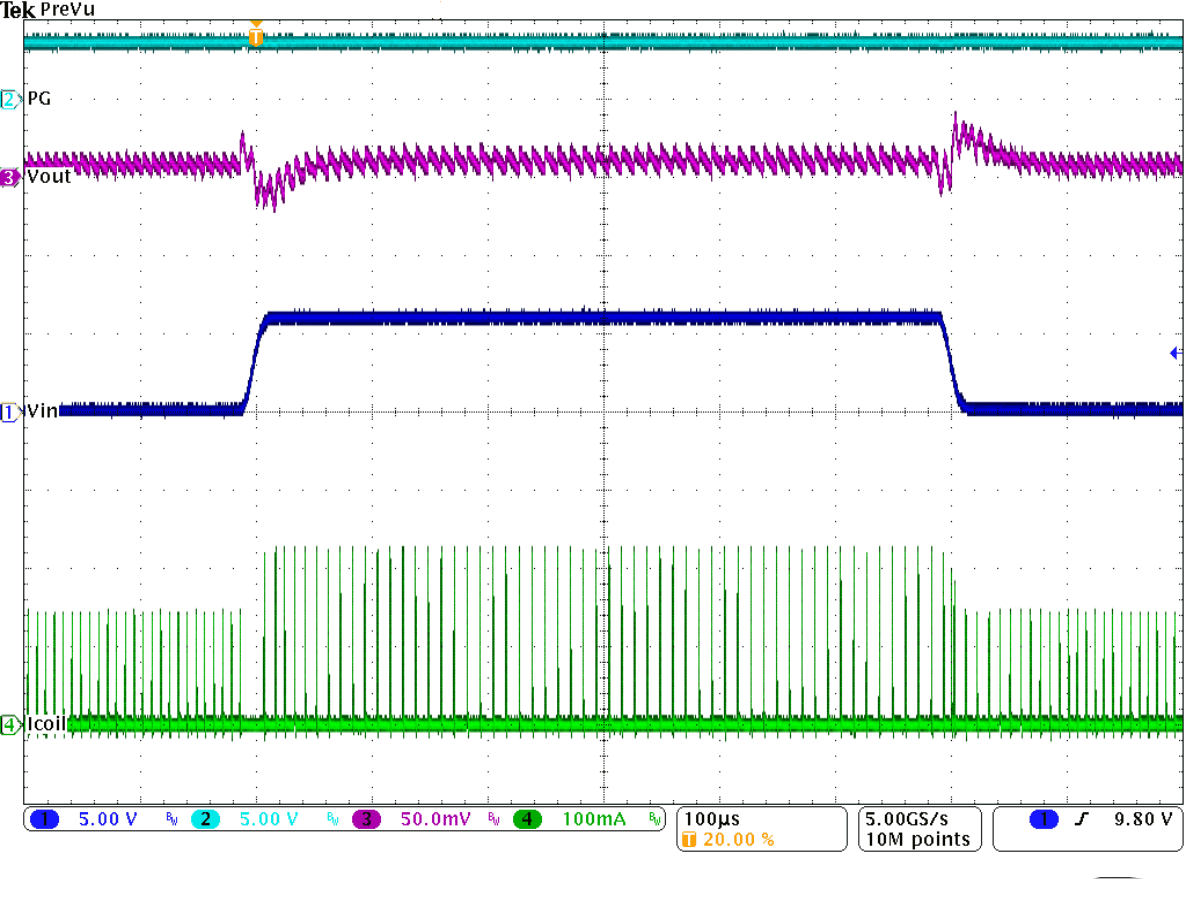Figure 39. Line Transient Response, Power Save Mode, VIN (6 V to 12 V), IOUT = 10 mA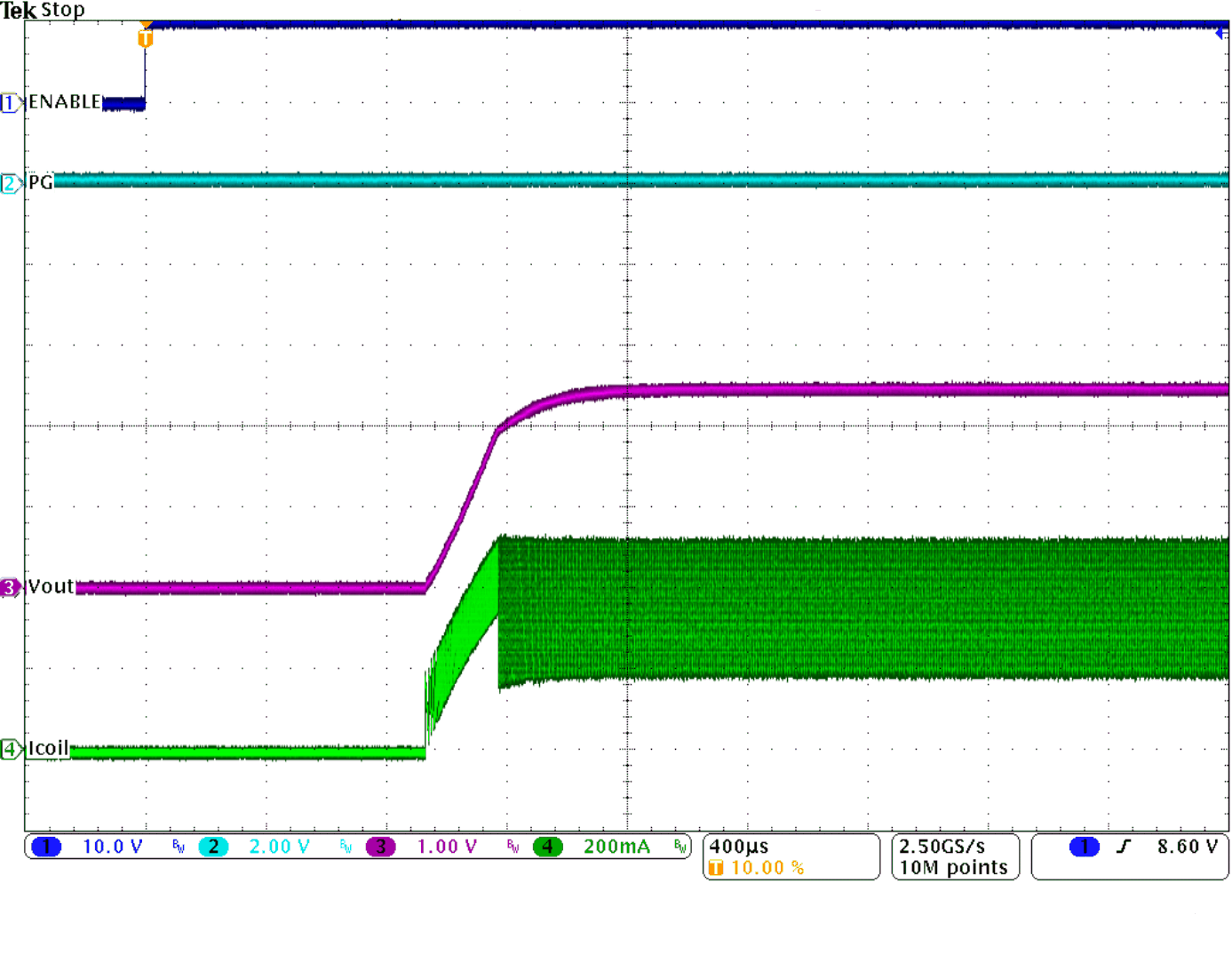Figure 41. Start-Up Current Limit, RLOAD = 6.6 Ω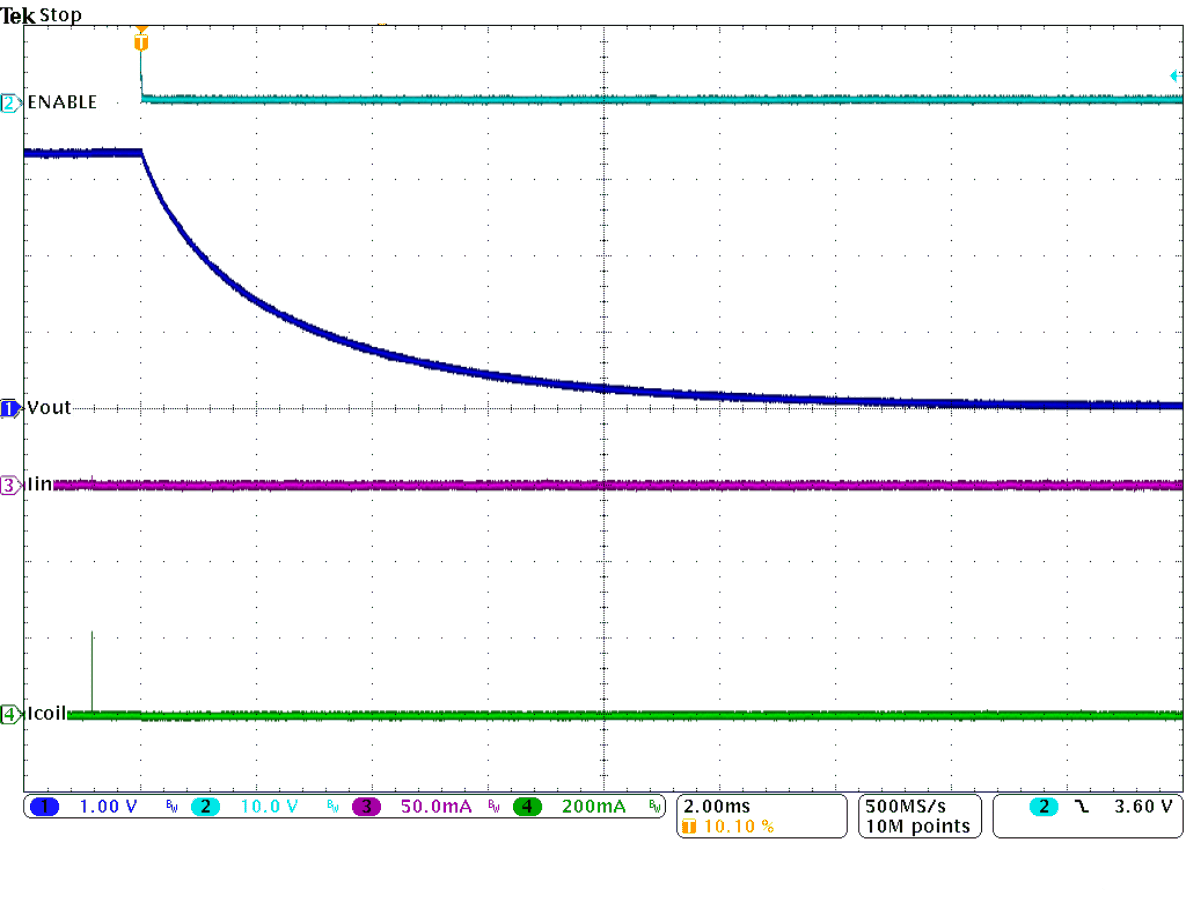Figure 43. Output Discharge Function (No Load)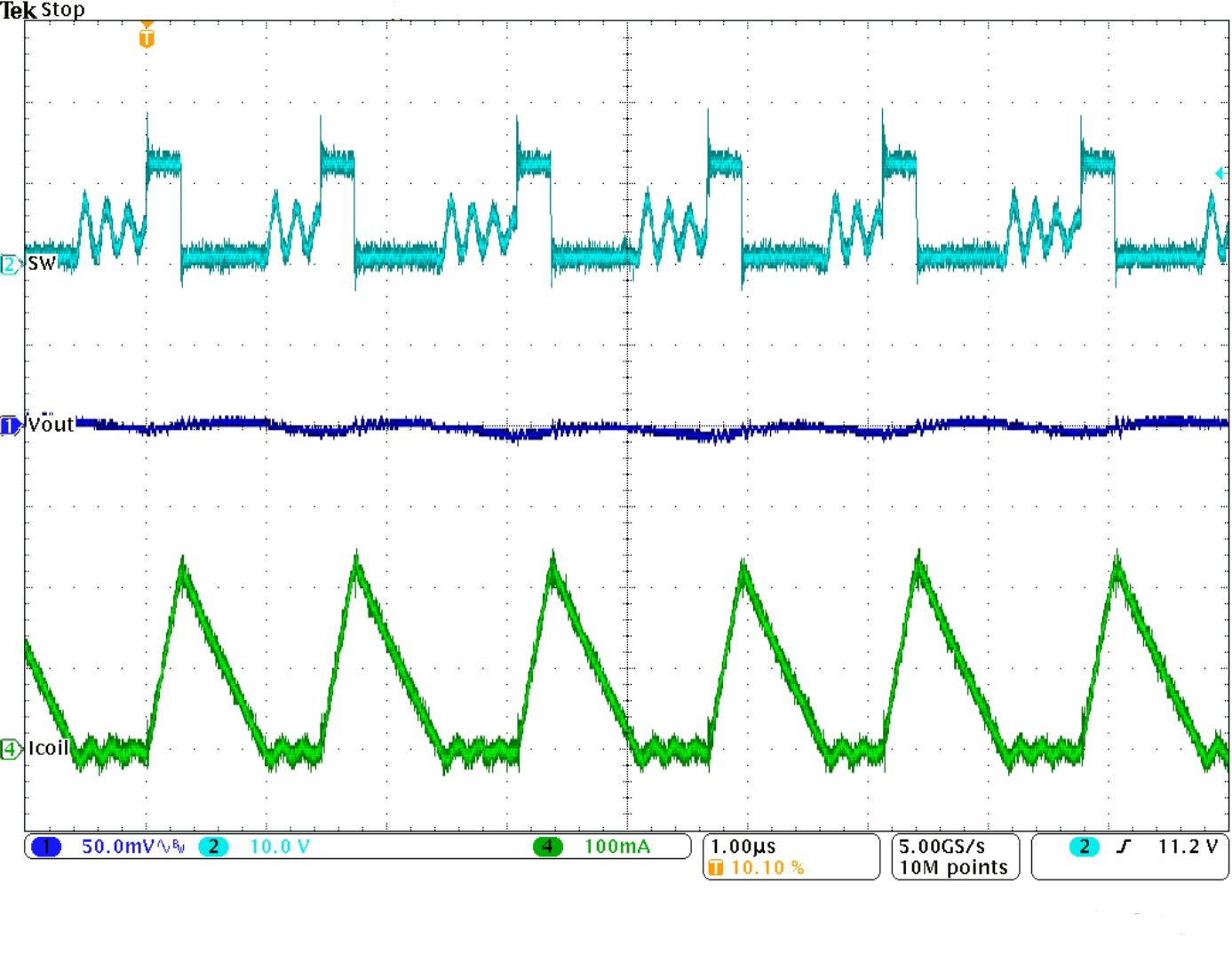Figure 45. Typical Operation in Power Save Mode, IOUT = 75 mA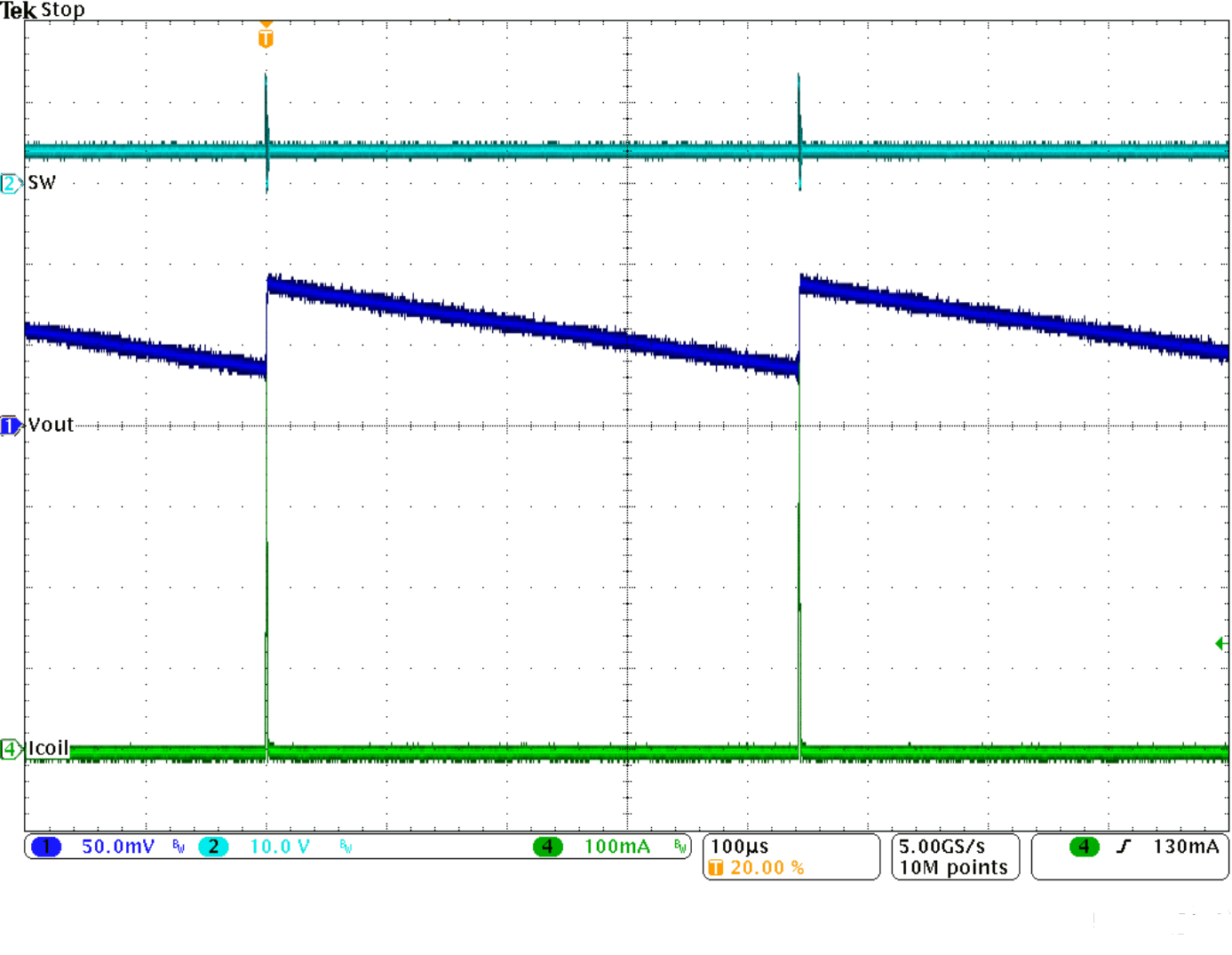Figure 47. Typical Operation in Sleep Mode, IOUT = 1 mA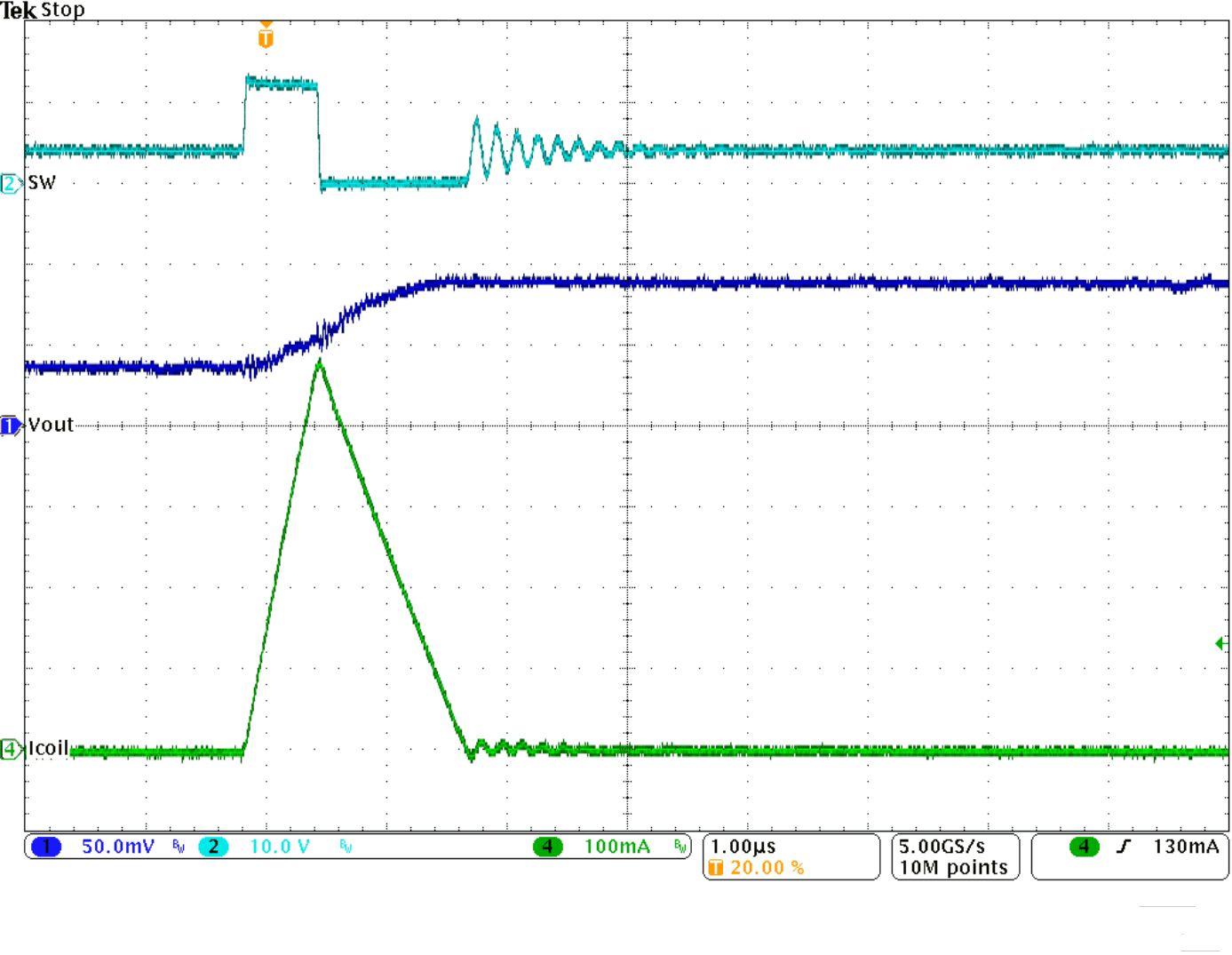Figure 49. Typical Operation in Sleep Mode, IOUT = 1 mA (Single Pulse)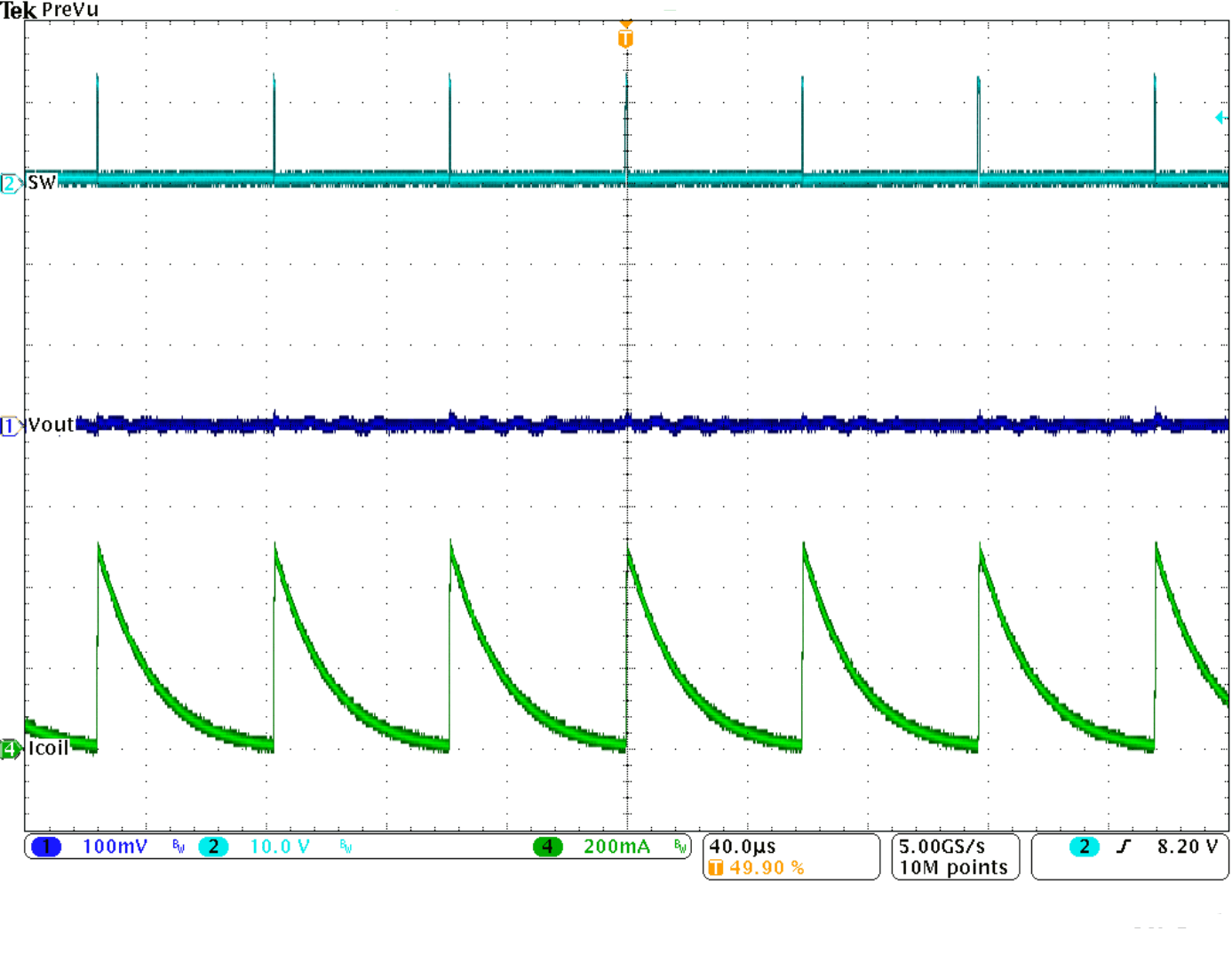Figure 51. Short Circuit From Start-Up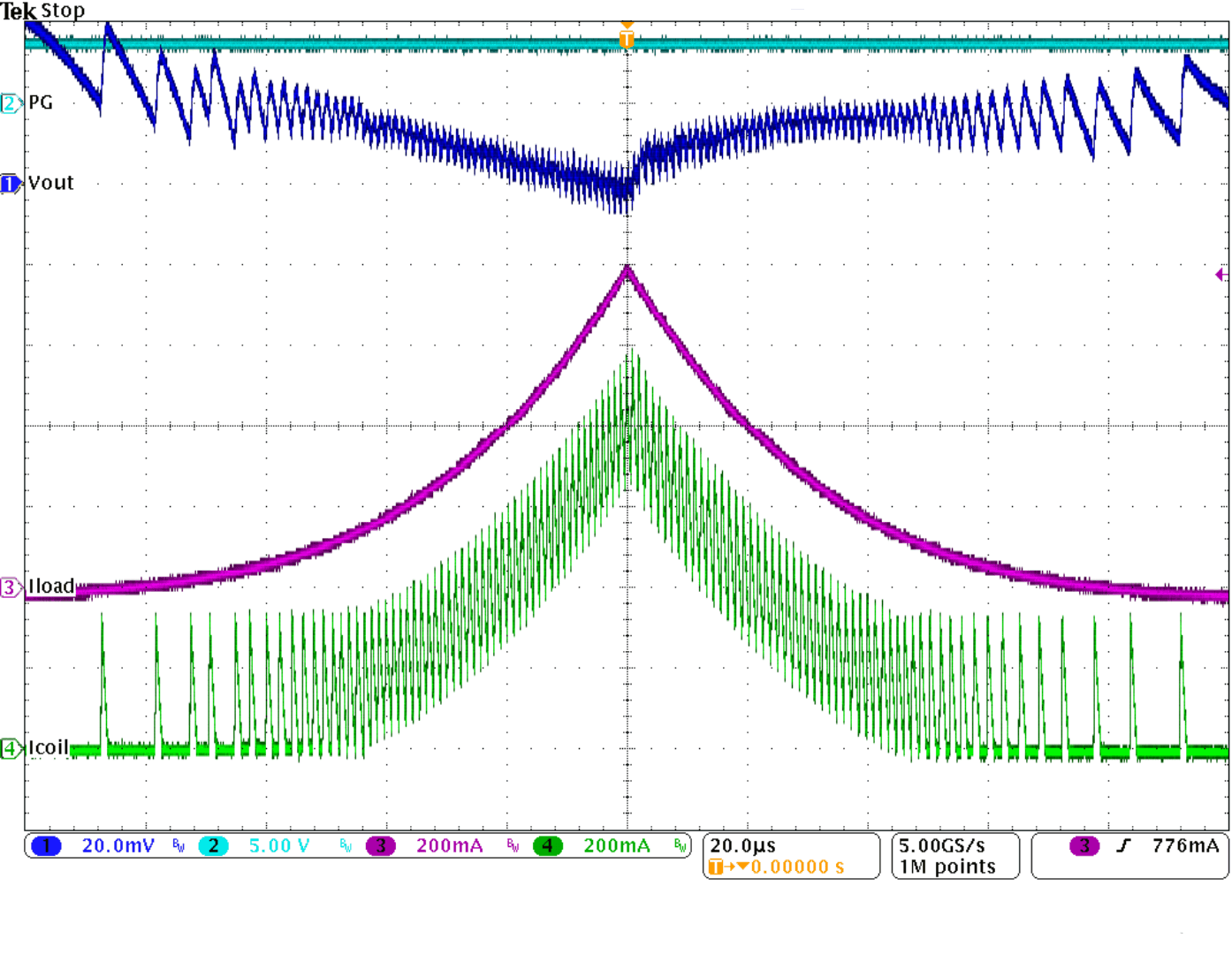Figure 53. Triangular Load Sweep With Mode Transitions (Power Save Mode - PWM Mode - Power Save Mode), VIN = 24 VFigure 10. Efficiency vs Input Voltage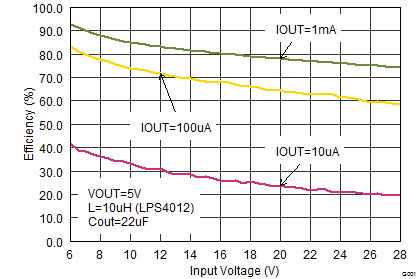Figure 12. Efficiency vs Input Voltage (Sleep Mode)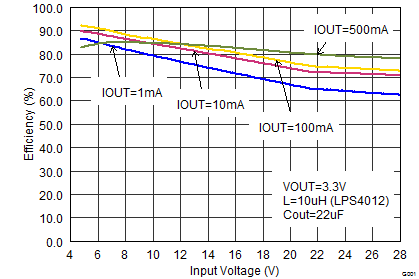Figure 14. Efficiency vs Input Voltage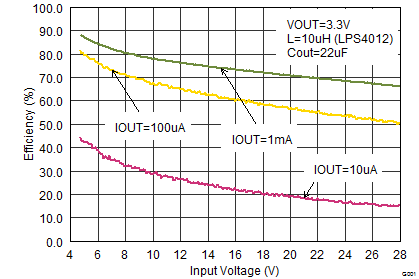Figure 16. Efficiency vs Input Voltage (Sleep Mode)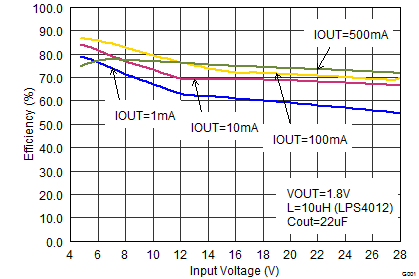Figure 18. Efficiency vs Input Voltage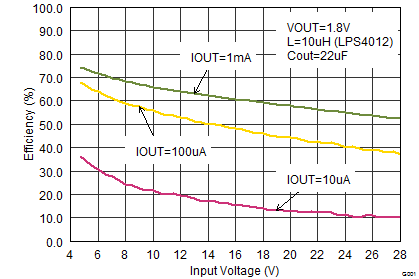Figure 20. Efficiency vs Input Voltage (Sleep Mode)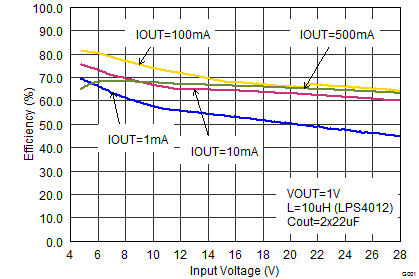Figure 22. Efficiency vs Input Voltage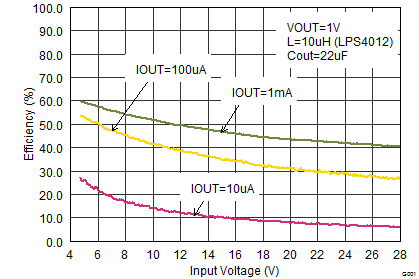Figure 24. Efficiency vs Input Voltage (Sleep Mode)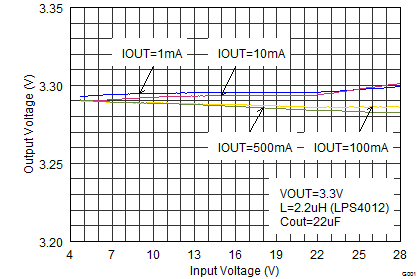Figure 26. Output Voltage Accuracy (Line Regulation)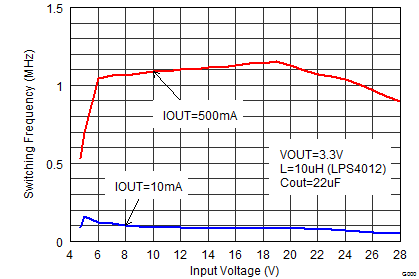Figure 28. Switching Frequency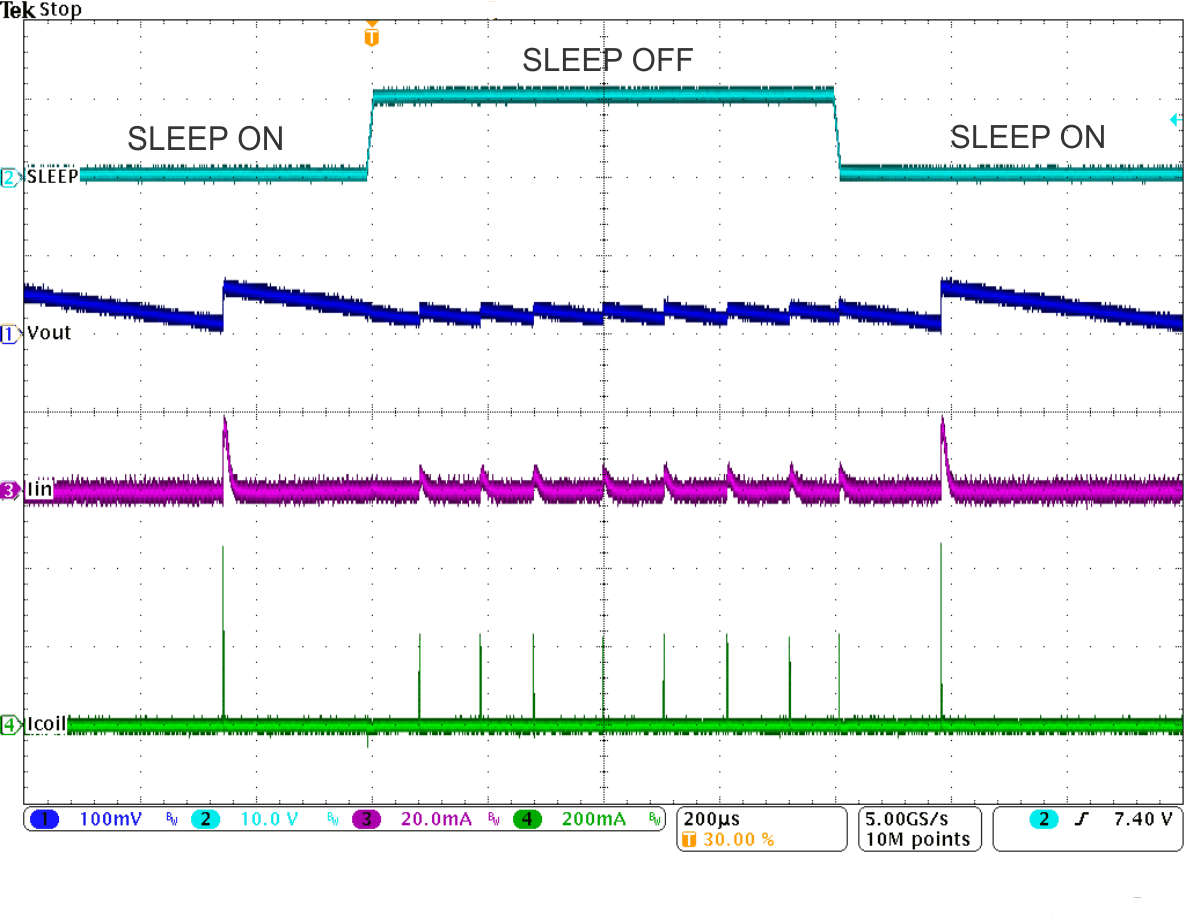Figure 30. Sleep Mode Entry/Exit, IOUT = 1 mAFigure 32. Load Transient Response, PWM Mode, IOUT (200 mA to 500 mA)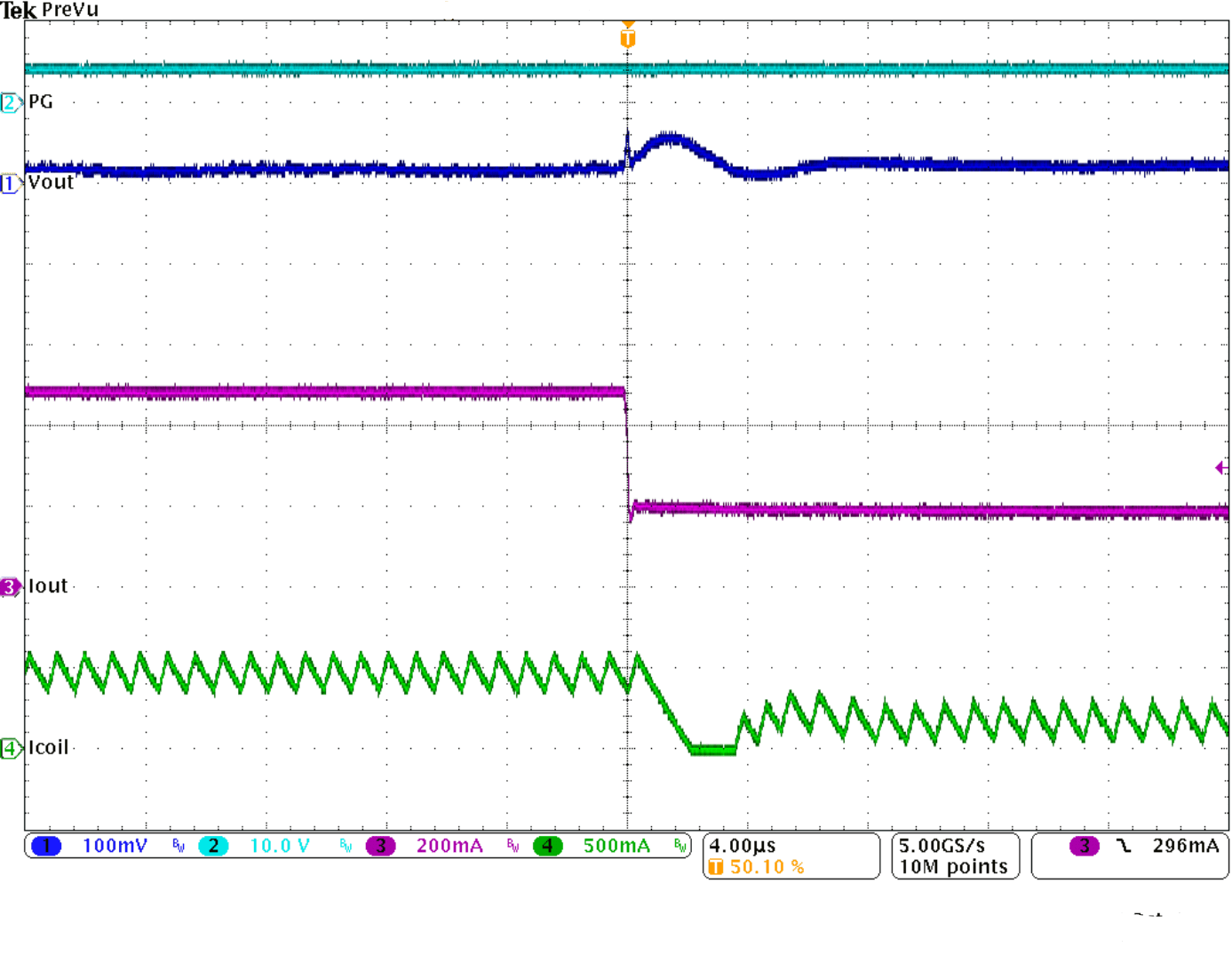Figure 34. Load Transient Response, PWM Mode, IOUT (200 mA to 500 mA), Falling Edge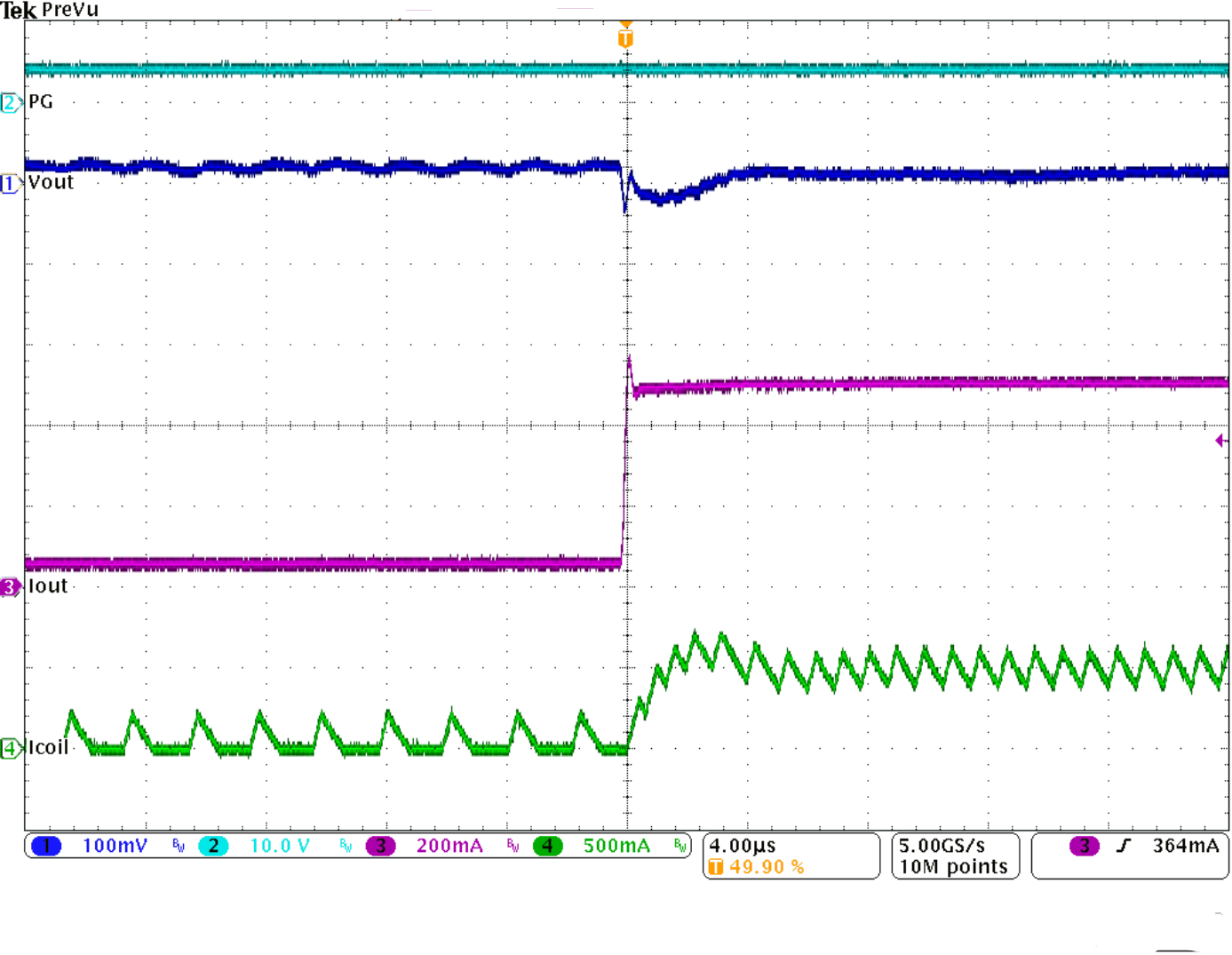Figure 36. Load Transient Response, Power Save Mode, IOUT (50 mA to 500 mA), Rising Edge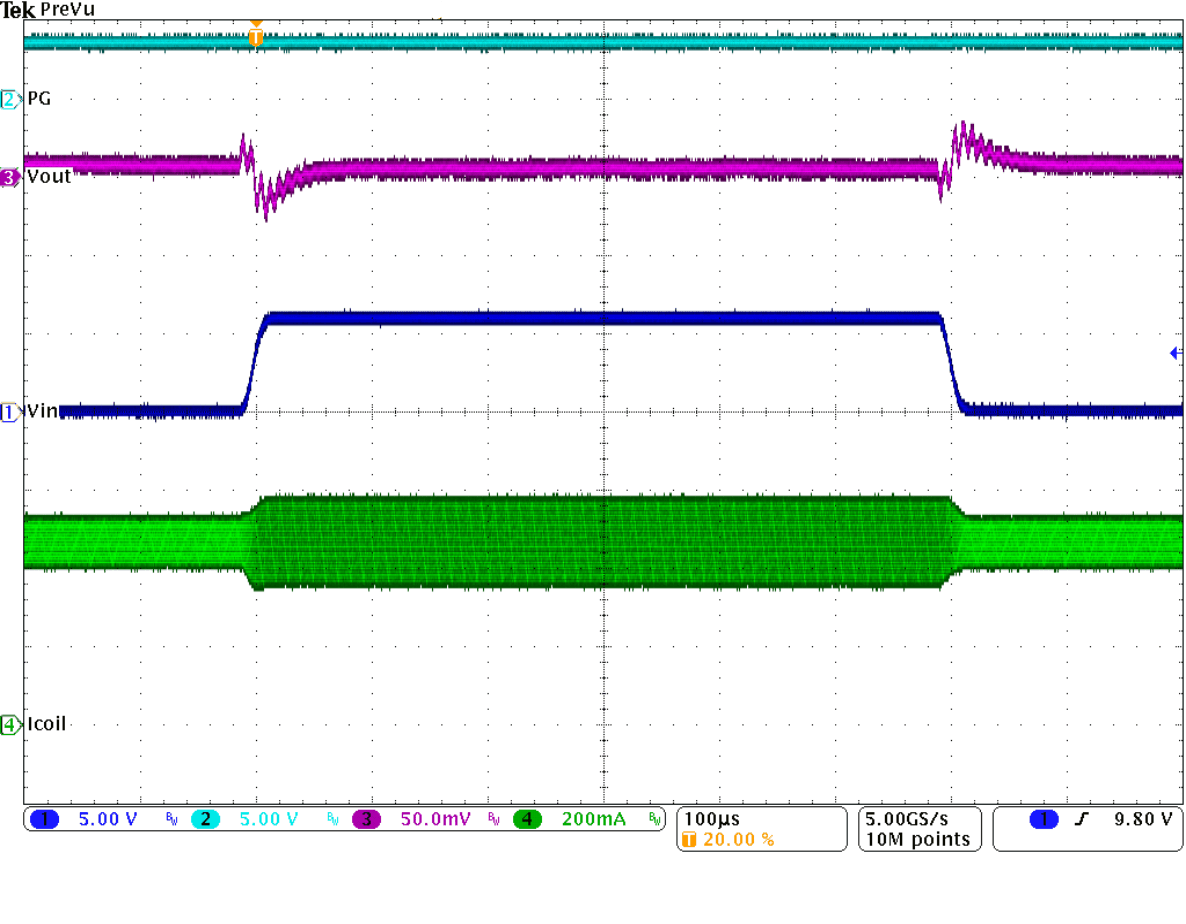Figure 38. Line Transient Response, PWM Mode, VIN (6 V to 12 V), IOUT = 500 mA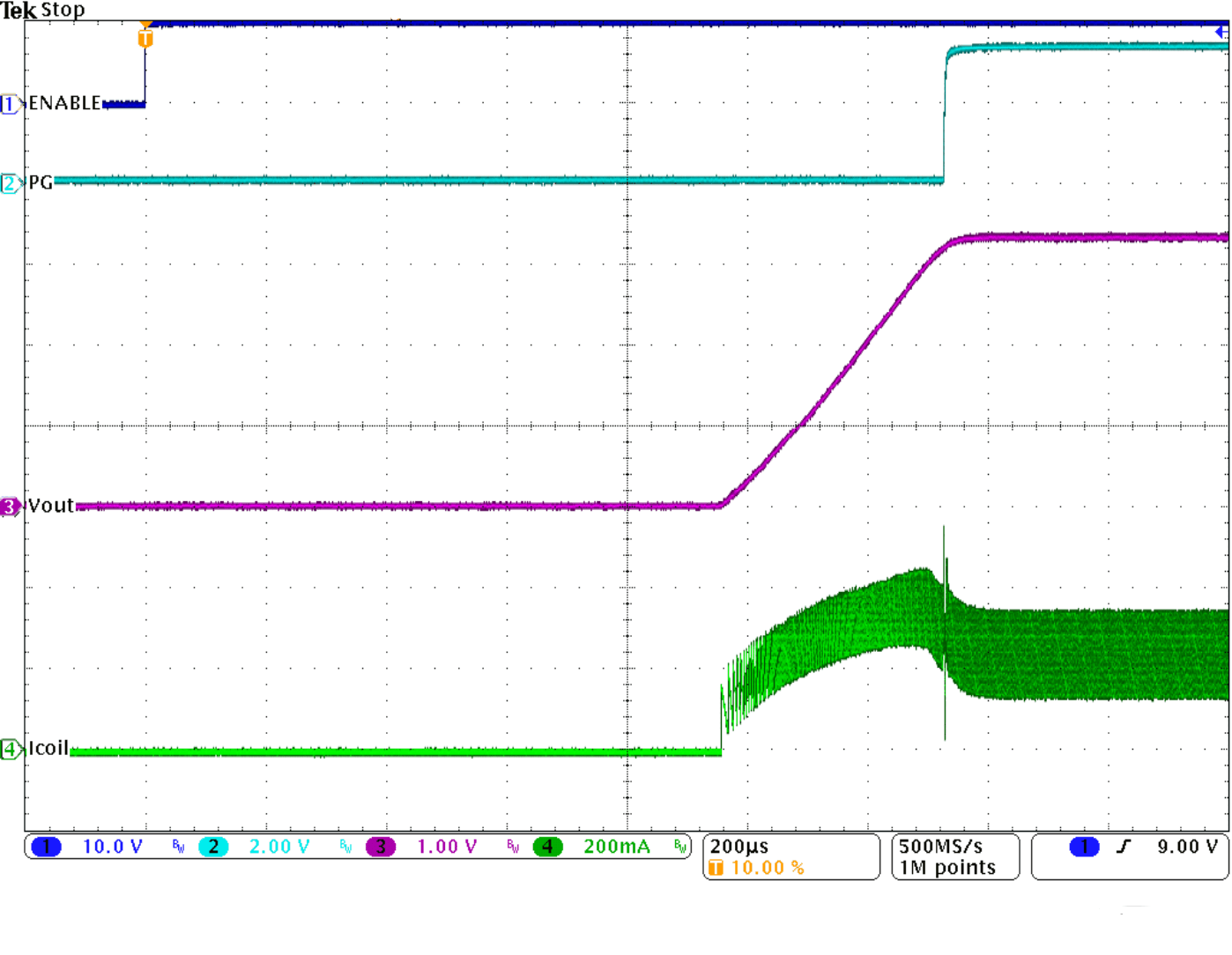Figure 40. Start-Up (PWM Mode), IOUT = 250 mA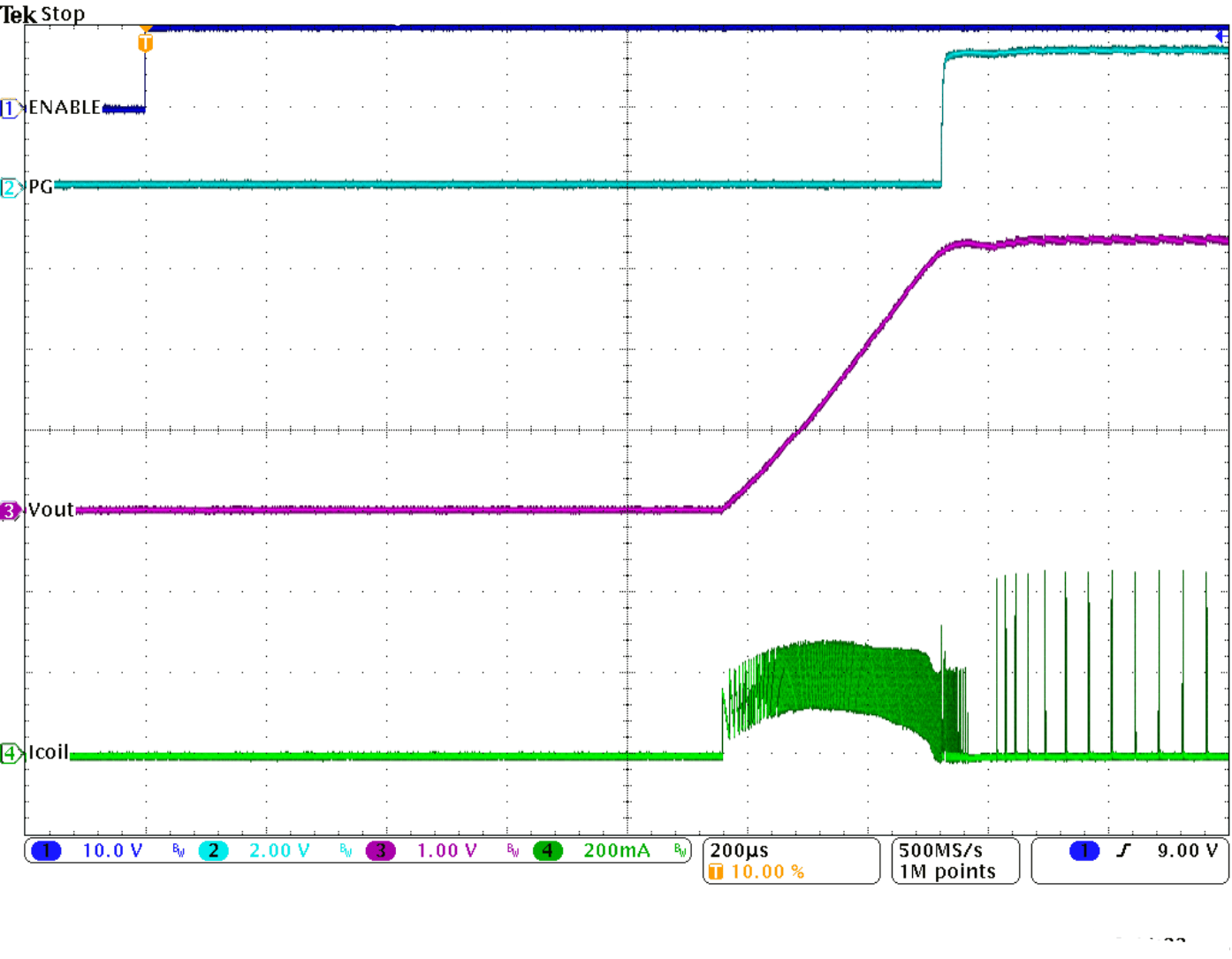Figure 42. Start-Up (Sleep Mode), IOUT = 10 mA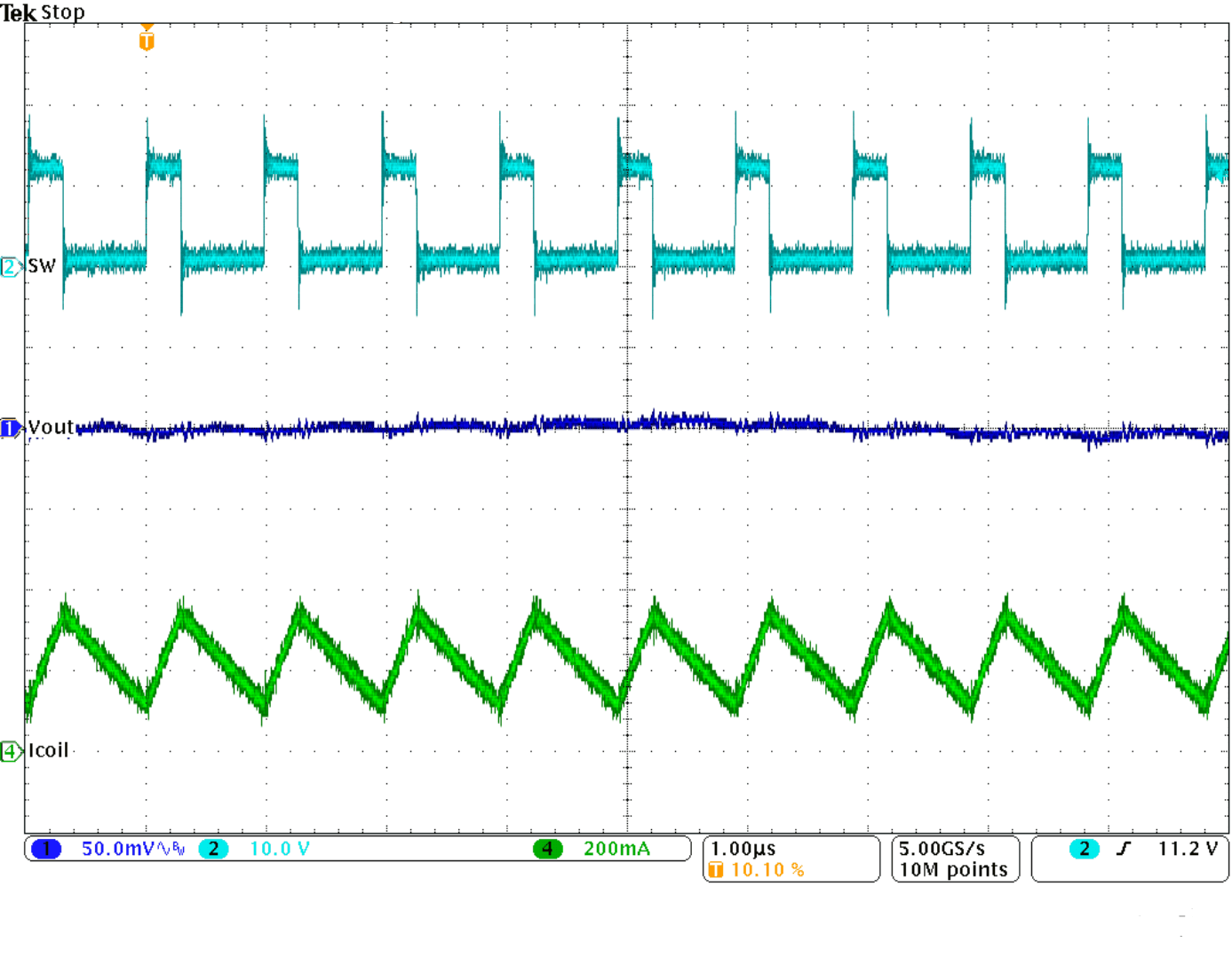Figure 44. Typical Operation in PWM Mode, IOUT = 250 mA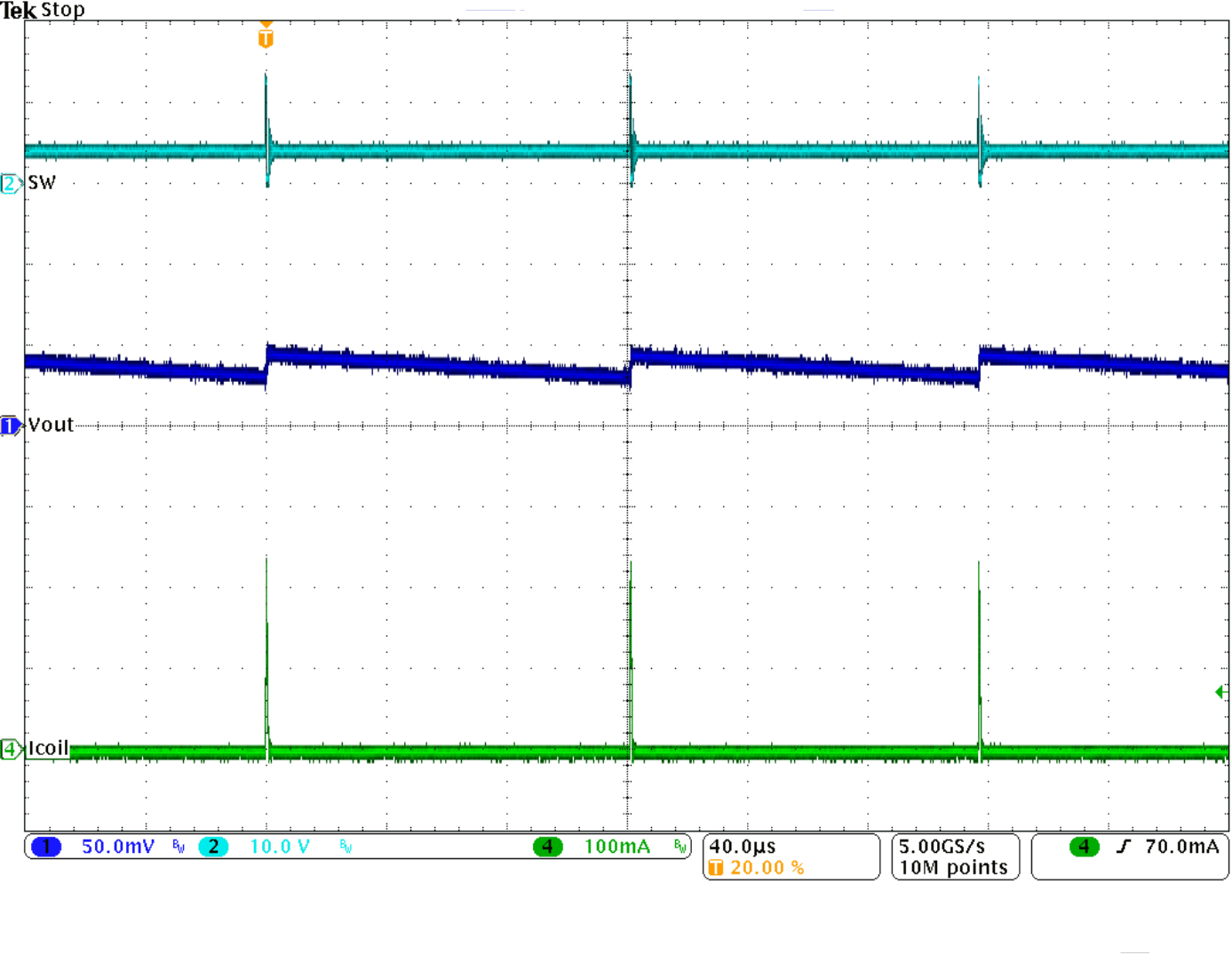Figure 46. Typical Operation in Power Save Mode, IOUT = 1 mA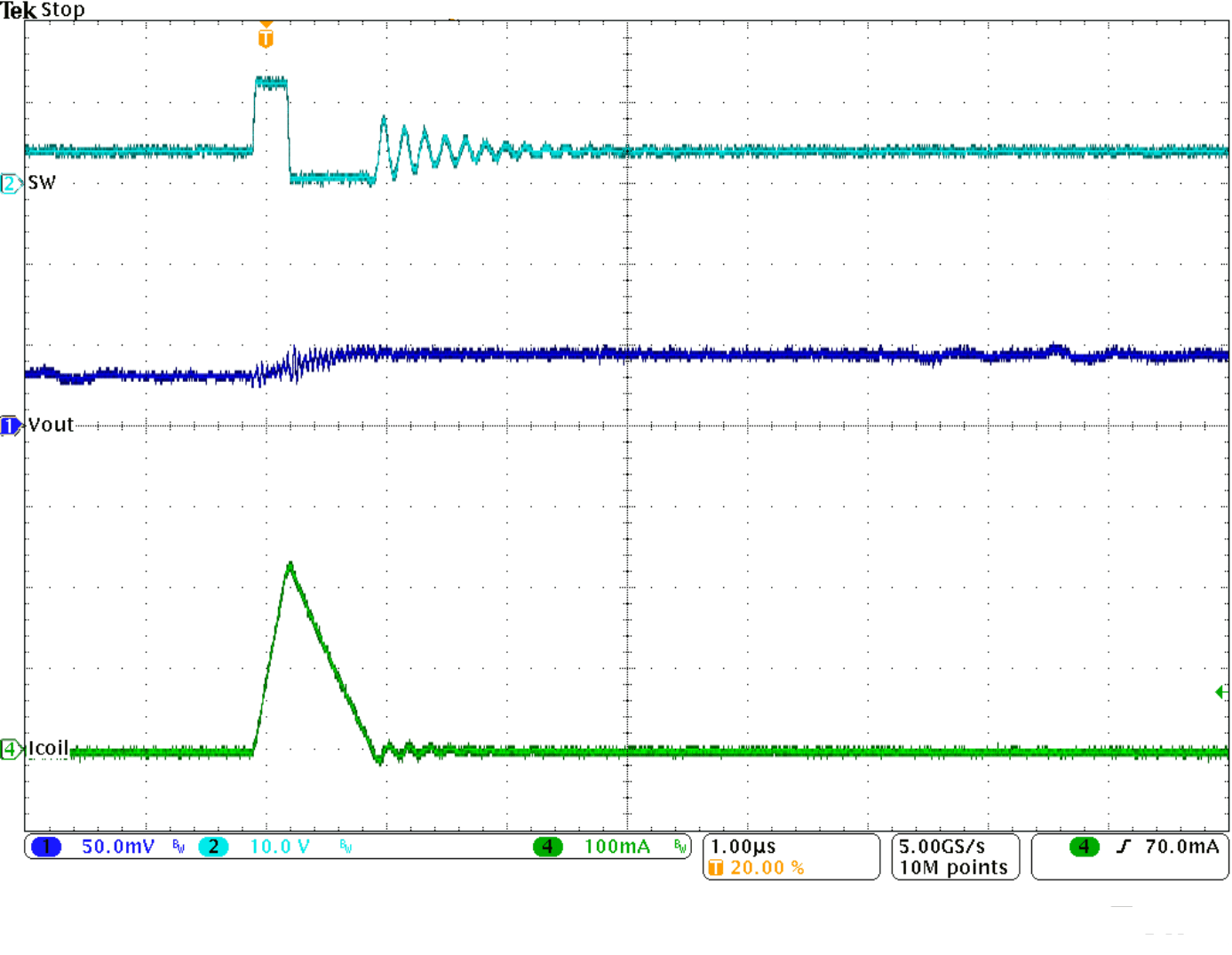Figure 48. Typical Operation in Power Save Mode, IOUT = 1 mA (Single Pulse)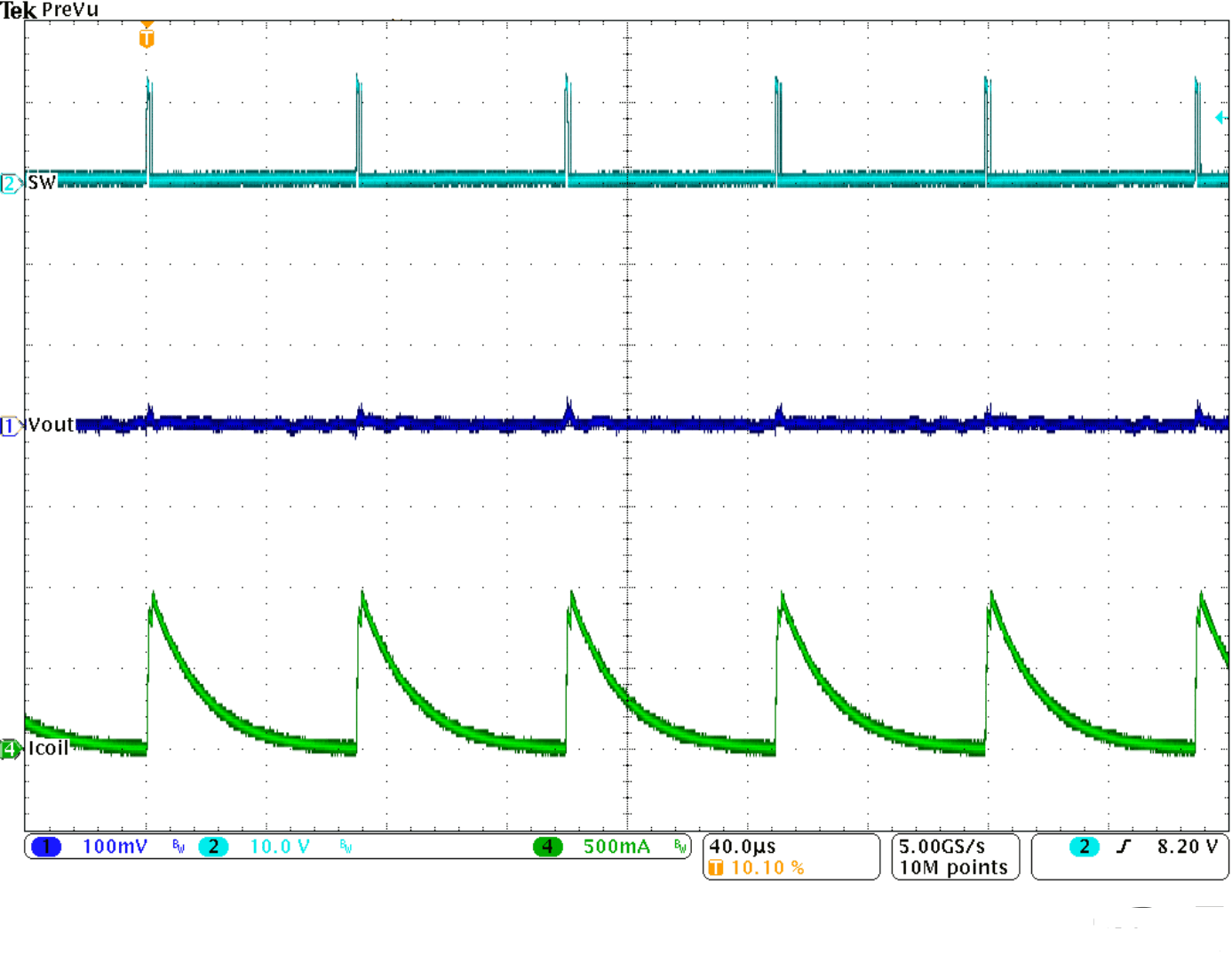Figure 50. Short Circuit While Running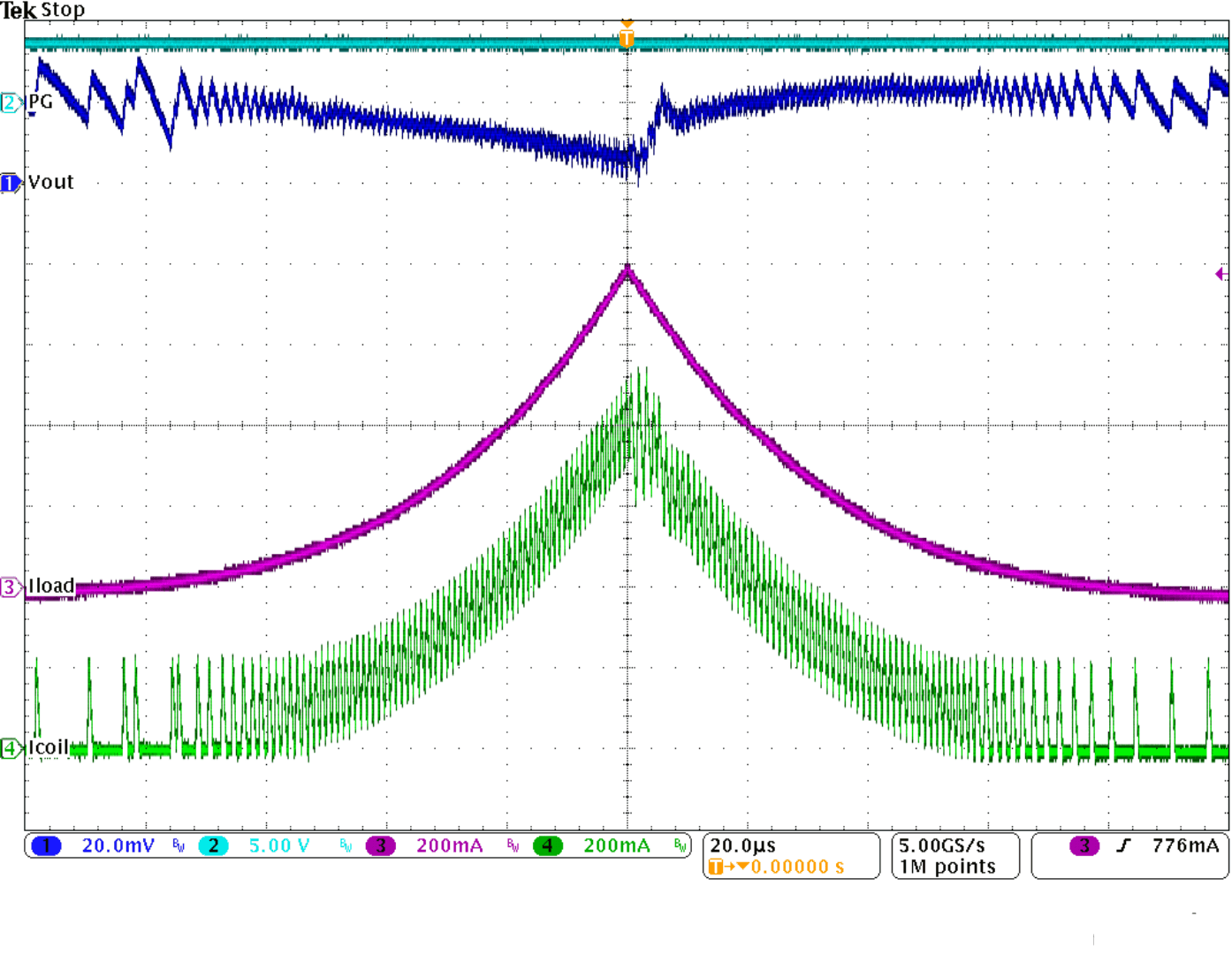Figure 52. Triangular Load Sweep With Mode Transitions (Power Save Mode - PWM Mode - Power Save Mode)

### 9.3 System Examples

#### 9.3.1 Microcontroller Power Supply

The TPS6217x can be used advantageously as the power supply rail for microcontrollers with low current power save modes. Figure 54 shows the connection of TPS62177 to the Tiva C Series TM4C123x ARM Cortex™ - M4 MCUs (TM4C123x MCUs), using its hibernate mode signal to control sleep mode operation. More information is found in the Application Report, Powering Tiva™ C Series Microcontrollers Using the High Efficiency DCS-Control™ Topology (SPMA066).

spacing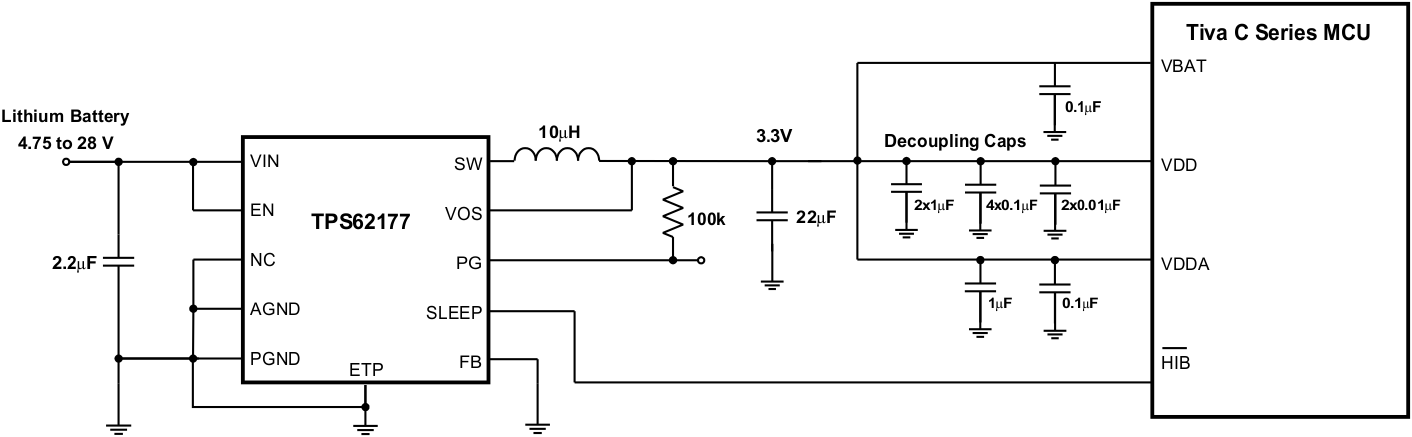Figure 54. Microcontroller Power Supply With Sleep Mode

spacing

#### 9.3.2 Inverting Power Supply

The TPS6217x can be used as inverting power supply by rearranging external circuitry as shown in Figure 55. As the former GND node now represents a voltage level below system ground, the voltage difference between VIN and VOUT must be limited to the maximum operating voltage of 28 V.

spacing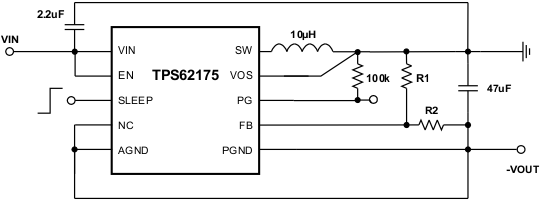Figure 55. Inverting Buck-Boost Converter

spacing

More information about using TPS62175 as inverting buck-boost converter can be found in the Application Note, Using the TPS62175 in an Inverting Buck Boost Topology (SLVA542).

#### 9.3.3 TPS62175 Adjustable Output Voltages

The following example circuits show typical schematics for commonly used output voltage values using the adjustable device version TPS62175.

#### 9.3.3.1 5-V / 0.5-A Power Supply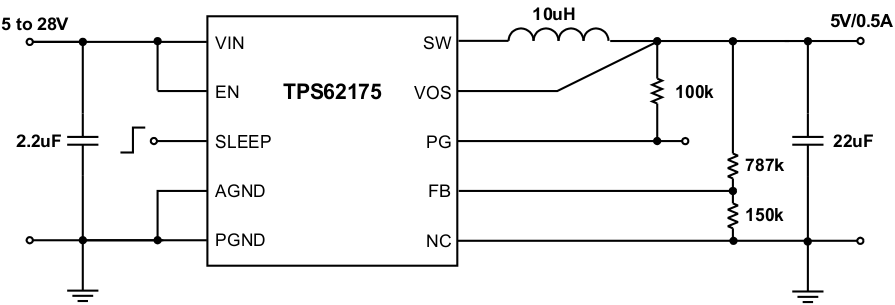Figure 56. 5-V / 0.5-A Power Supply

#### 9.3.3.2 2.5-V / 0.5-A Power Supply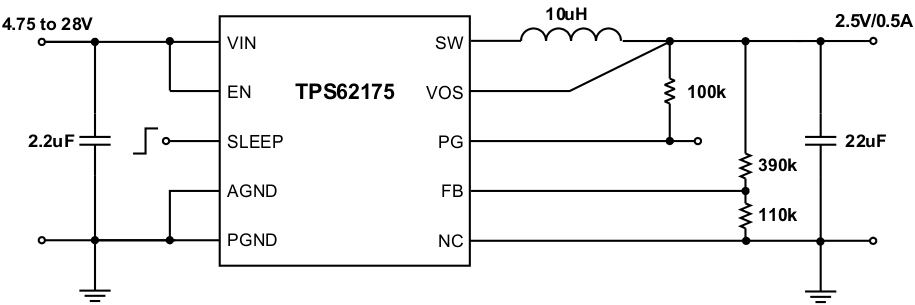Figure 57. 2.5-V / 0.5-A Power Supply

#### 9.3.3.3 1.8-V / 0.5-A Power Supply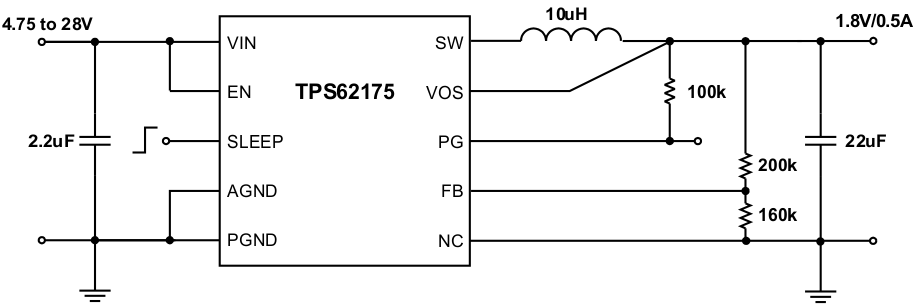Figure 58. 1.8-V / 0.5-A Power Supply

#### 9.3.3.4 1.2-V / 0.5-A Power Supply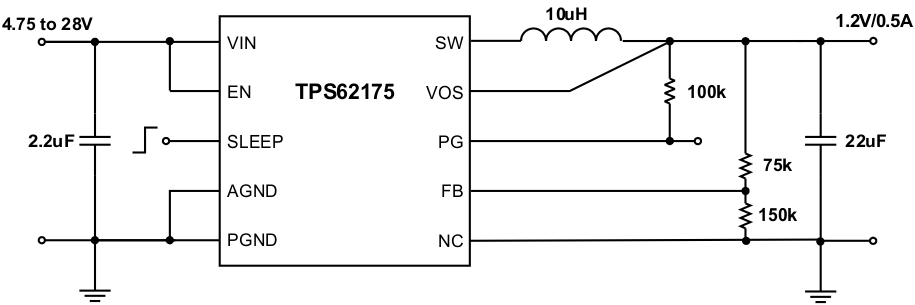Figure 59. 1.2-V / 0.5-A Power Supply

#### 9.3.3.5 1-V / 0.5-A Power Supply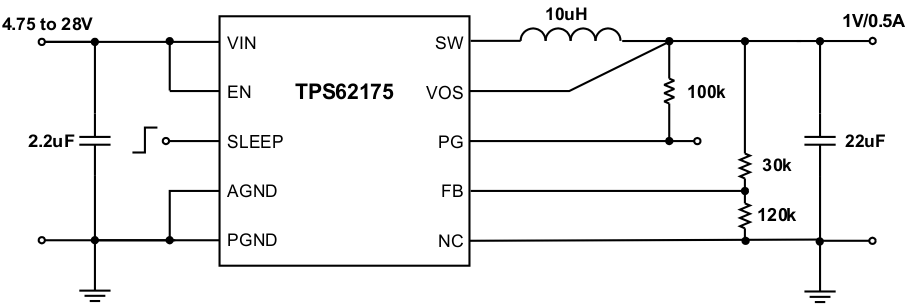Figure 60. 1-V / 0.5-A Power Supply

#### 9.3.4 TPS62177 Fixed 3.3-V / 0.5-A Power Supply

The following example circuit shows the typical schematic for fixed output voltage using the device version TPS62177.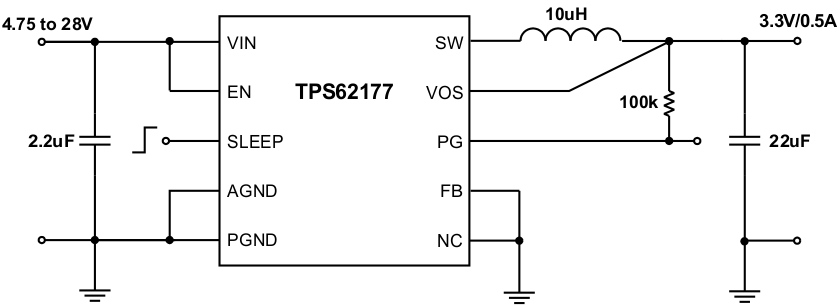Figure 61. 3.3-V / 0.5-A Power Supply